# 9. Exploring Relationships Between Variables

The online version of the open-access textbook Minimalist Data Wrangling with Python by Marek Gagolewski is, and will remain, freely available for everyone’s enjoyment (also in PDF). Any bug/typos reports/fixes are appreciated. Although available online, this is a whole course; it should be read from the beginning to the end. In particular, refer to the Preface for general introductory remarks.

Let us consider the National Health and Nutrition Examination Survey (NHANES study) excerpt once again:

body = pd.read_csv("https://raw.githubusercontent.com/gagolews/" +
comment="#")
body = body.to_numpy()  # data frames will be covered later
body.shape
## (4221, 7)
body[:6, :]  # 6 first rows, all columns
## array([[ 97.1, 160.2,  34.7,  40.8,  35.8, 126.1, 117.9],
##        [ 91.1, 152.7,  33.5,  33. ,  38.5, 125.5, 103.1],
##        [ 73. , 161.2,  37.4,  38. ,  31.8, 106.2,  92. ],
##        [ 61.7, 157.4,  38. ,  34.7,  29. , 101. ,  90.5],
##        [ 55.4, 154.6,  34.6,  34. ,  28.3,  92.5,  73.2],
##        [ 62. , 144.7,  32.5,  34.2,  29.8, 106.7,  84.8]])


We thus have n=4221 participants and 7 different features describing them, in this order:

1. weight (kg),

2. standing height (cm),

3. upper arm length (cm),

4. upper leg length (cm),

5. arm circumference (cm),

6. hip circumference (cm),

7. waist circumference (cm).

We expect the data in columns to be related to each other (e.g., a taller person usually tends to weight more). This is why in this chapter we are interested in quantifying the degree of association and modelling functional relationships between the variables as well as finding new interesting combinations thereof.

## 9.1. Measuring Correlation

Scatterplots let us identify some simple patterns or structure in data. Figure 7.4 indicates that higher hip circumferences tend to occur more often together with higher arm circumferences and that the latter does not really tell us anything about height.

Let us explore some basic means for measuring (expressing as a single number) the degree of association between a set of pairs of points.

### 9.1.1. Pearson’s Linear Correlation Coefficient

First, the Pearson’s linear correlation coefficient:

$r(\boldsymbol{x}, \boldsymbol{y}) = \frac{1}{n} \sum_{i=1}^n \frac{x_i - \bar{x}}{s_{x}} \, \frac{y_i - \bar{y}}{s_{y}},$

with $$s_x, s_y$$ denoting the standard deviations and $$\bar{x}, \bar{y}$$ being the means of $$\boldsymbol{x}=(x_1,\dots,x_n)$$ and $$\boldsymbol{y}=(y_1,\dots,y_n)$$, respectively.

Note

Look carefully: we are computing pairwise products of standardised versions of the two vectors. It is a normalised measure of how they vary together (co-variance).

(*) Furthermore, in Section 9.3.1 we mention that $$r$$ is the cosine of the angle between centred and normalised versions of the vectors.

Here is how we can compute it manually:

x = body[:, 4]  # arm circumference
y = body[:, 5]  # hip circumference
x_std = (x-np.mean(x))/np.std(x)
y_std = (y-np.mean(y))/np.std(y)
np.sum(x_std*y_std)/len(x)
## 0.8680627457873239


And here is a built-in function that implements the same formula:

scipy.stats.pearsonr(x, y)
## 0.8680627457873241


Important

Basic properties of Pearson’s r include:

1. $$r(\boldsymbol{x}, \boldsymbol{y}) = r(\boldsymbol{y}, \boldsymbol{x})$$ (symmetric);

2. $$|r(\boldsymbol{x}, \boldsymbol{y})| \le 1$$ (bounded from below by -1 and from above by 1);

3. $$r(\boldsymbol{x}, \boldsymbol{y})=1$$ if and only if $$\boldsymbol{y}=a\boldsymbol{x}+b$$ for some $$a>0$$ and $$b$$, (reaches the maximum when one variable is an increasing linear function of the other one);

4. $$r(\boldsymbol{x}, -\boldsymbol{y})=-r(\boldsymbol{x}, \boldsymbol{y})$$ (negative scaling (reflection) of one variable changes the sign of the coefficient);

5. $$r(\boldsymbol{x}, a\boldsymbol{y}+b)=r(\boldsymbol{x}, \boldsymbol{y})$$ for any $$a>0$$ and $$b$$ (invariant to translation and scaling of inputs that does not change the sign of elements).

To get more insight, below we shall illustrate some interesting correlations using the following function that draws a scatter plot and prints out Pearson’s r (and Spearman’s ρ which we discuss in Section 9.1.4 – let us ignore it by then):

def plot_corr(x, y):
r = scipy.stats.pearsonr(x, y)
ρ = scipy.stats.spearmanr(x, y)
plt.plot(x, y, "o", label=f"r = {r:.3}\nρ = {ρ:.3}")
plt.legend()
pass


#### 9.1.1.1. Perfect Linear Correlation

The aforementioned properties imply that $$r(\boldsymbol{x}, \boldsymbol{y})=-1$$ if and only if $$\boldsymbol{y}=a\boldsymbol{x}+b$$ for some $$a<0$$ and $$b$$ (reaches the minimum when one variable is a decreasing linear function of the other one) Furthermore, a variable is trivially perfectly correlated with itself $$r(\boldsymbol{x}, \boldsymbol{x})=1$$.

Hence, we get perfect linear correlation (-1 or 1) when one variable is a scaled and shifted version (linear function) of the other variable, see Figure 9.1.

np.random.seed(123)
x = np.random.rand(100)
plt.subplot(1, 2, 1)
plot_corr(x, -0.5*x+3)  # negative slope
plt.axis("equal")
plt.subplot(1, 2, 2)
plot_corr(x, 3*x+10)  # positive slope
plt.axis("equal")
plt.show()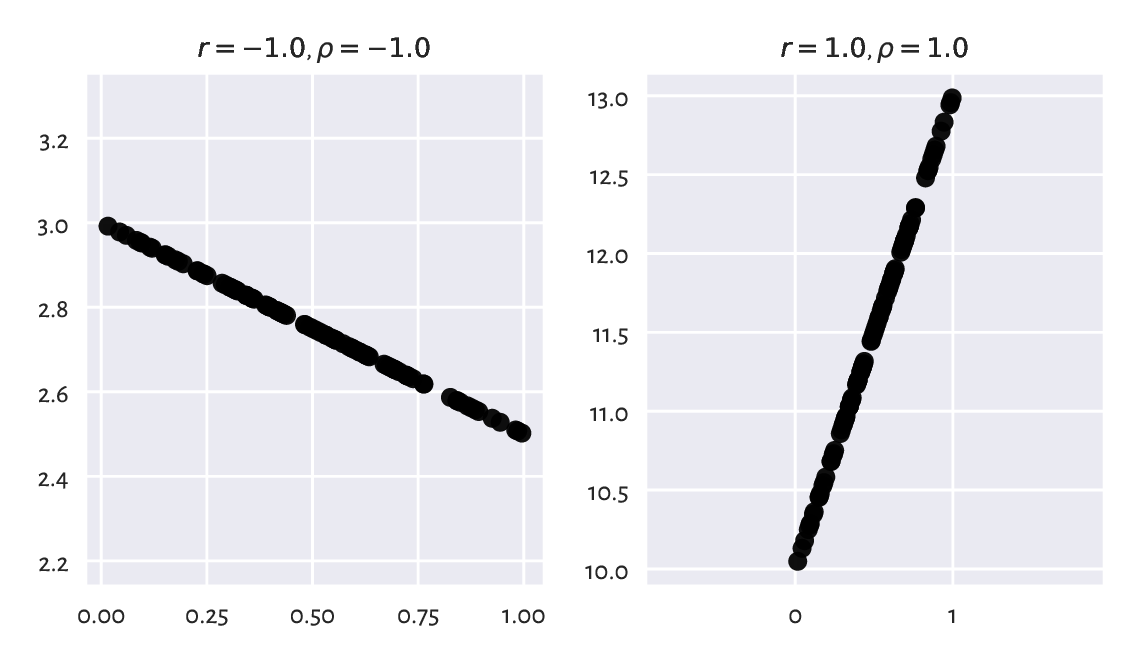Figure 9.1 Perfect linear correlation (negative and positive)

A negative correlation means that when one variable increases, the other one decreases (like: a car’s braking distance vs velocity).

#### 9.1.1.2. Strong Linear Correlation

Next, if two variables are more or less linear functions of themselves, the correlations will be close to -1 or 1, with the degree of association diminishing as the linear relationship becomes less and less present, see Figure 9.2.

np.random.seed(123)
x = np.random.rand(100)
y = 0.5*x
e = np.random.randn(len(x))  # random white noise (of mean 0)
plt.figure(figsize=(plt.rcParams["figure.figsize"], )*2)  # width=height
plt.subplot(2, 2, 1)
plot_corr(x, y)
plt.subplot(2, 2, 2)
plot_corr(x, y+0.05*e)  # add some noise
plt.subplot(2, 2, 3)
plot_corr(x, y+0.1*e)   # more noise
plt.subplot(2, 2, 4)
plot_corr(x, y+0.25*e)  # even more noise
plt.show()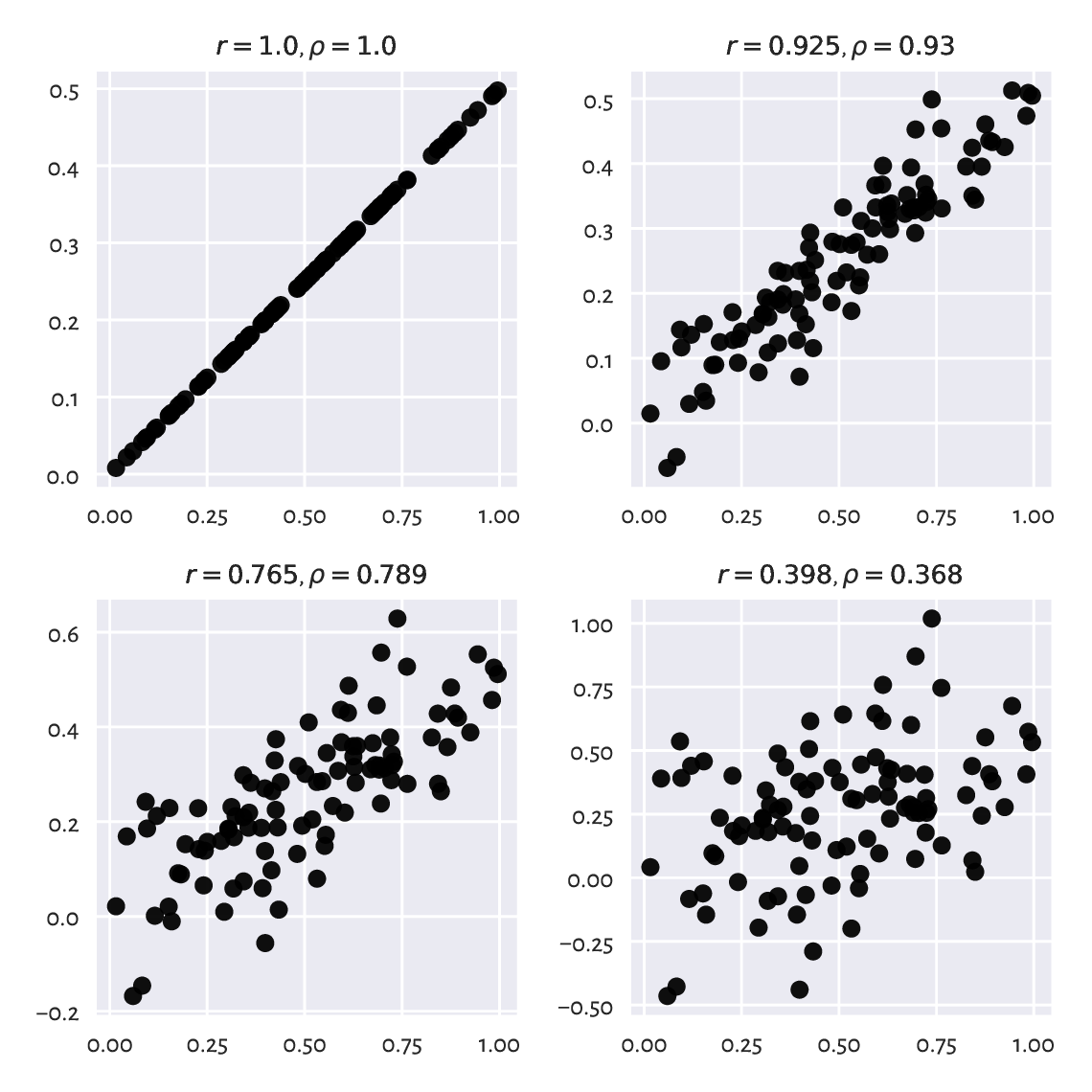Figure 9.2 Linear correlation coefficients for data with different amounts of noise

Notice again that the arm and hip circumferences enjoy quite high positive degree of linear correlation.

Exercise 9.1

Draw a series of similar plots but for the case of negatively correlated point pairs.

Important

As a rule of thumb, linear correlation degree of 0.9 or greater (or -0.9 or smaller) is quite decent. Between -0.8 and 0.8 we probably should not be talking about two variables being linearly correlated at all. Some textbooks are more lenient, but we have higher standards. In particular, it is not uncommon in social sciences to consider 0.6 a decent degree of correlation, but this is like building on sand. If a dataset at hand does not provide us with strong evidence, it is our ethical duty to refrain ourselves from publishing unjustified statements.

#### 9.1.1.3. No Linear Correlation Does Not Imply Independence

We should stress that correlation close to 0 does not necessarily mean that two variables are not related to each other, although for two independent variables we definitely expect the correlation coefficient be approximately equal to 0. Pearson’s r is a linear correlation coefficient, so we are only quantifying these types of relationships. See Figure 9.3 for an illustration of this fact.

np.random.seed(123)
plt.figure(figsize=(plt.rcParams["figure.figsize"], )*2)  # width=height
plt.subplot(2, 2, 1)
plot_corr(x, np.random.rand(100))  # independent (not correlated)
plt.subplot(2, 2, 2)
plt.subplot(2, 2, 3)
plot_corr(x, np.abs(2*x-1))  # another form of dependence
plt.subplot(2, 2, 4)
plot_corr(x, np.sin(10*np.pi*x))  # another
plt.show()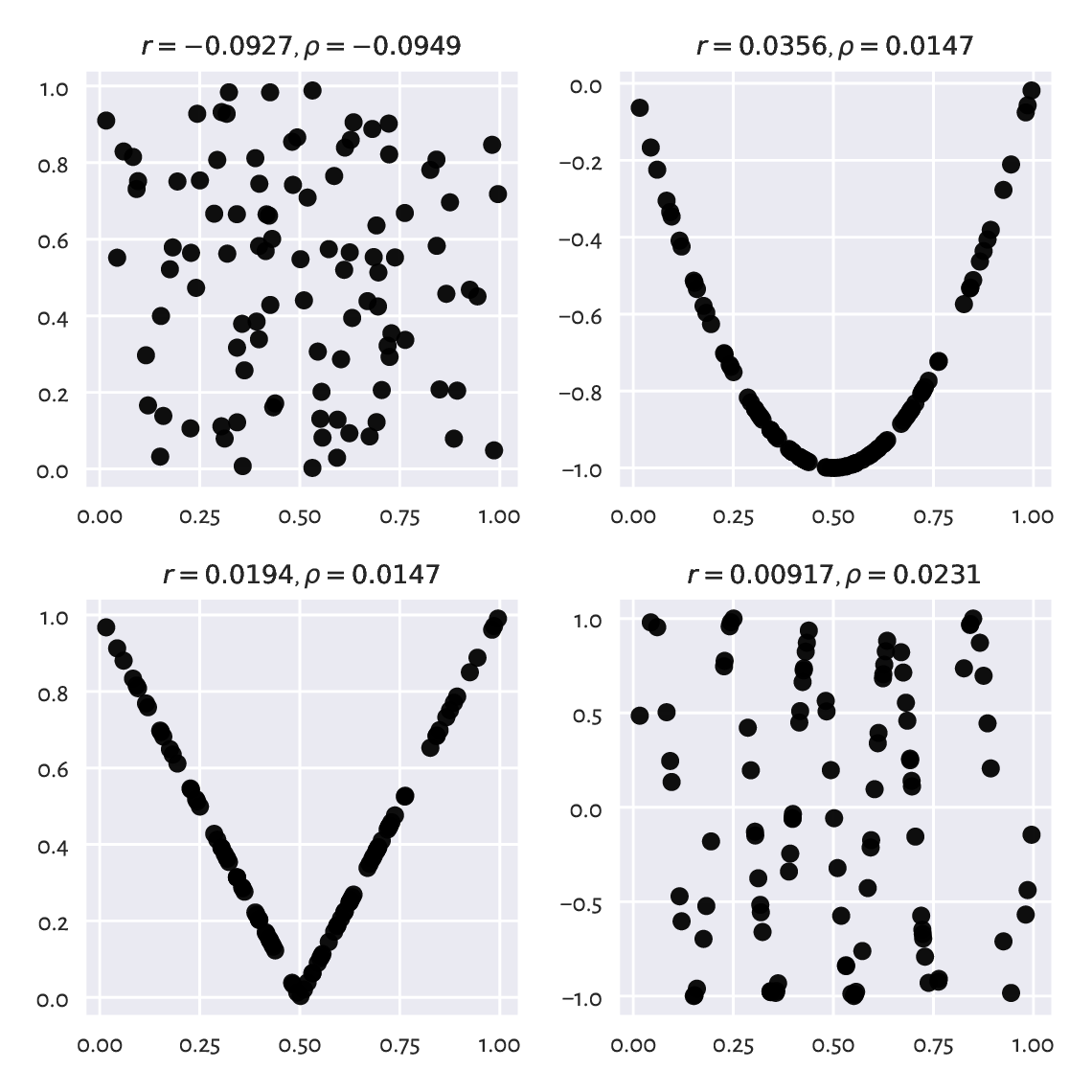Figure 9.3 Are all of these really uncorrelated?

#### 9.1.1.4. False Linear Correlations

What is more, sometimes we can detect false correlations – when data are functionally dependent, the relationship is not linear, but it kind of looks like linear. Refer to Figure 9.4 for some examples.

np.random.seed(123)
plt.figure(figsize=(plt.rcParams["figure.figsize"], )*2)  # width=height
plt.subplot(2, 2, 1)
plot_corr(x, np.sin(0.6*np.pi*x))
plt.subplot(2, 2, 2)
plot_corr(x, np.log(x+1))
plt.subplot(2, 2, 3)
plot_corr(x, np.exp(x**2))
plt.subplot(2, 2, 4)
plot_corr(x, 1/(x/2+0.2))
plt.show()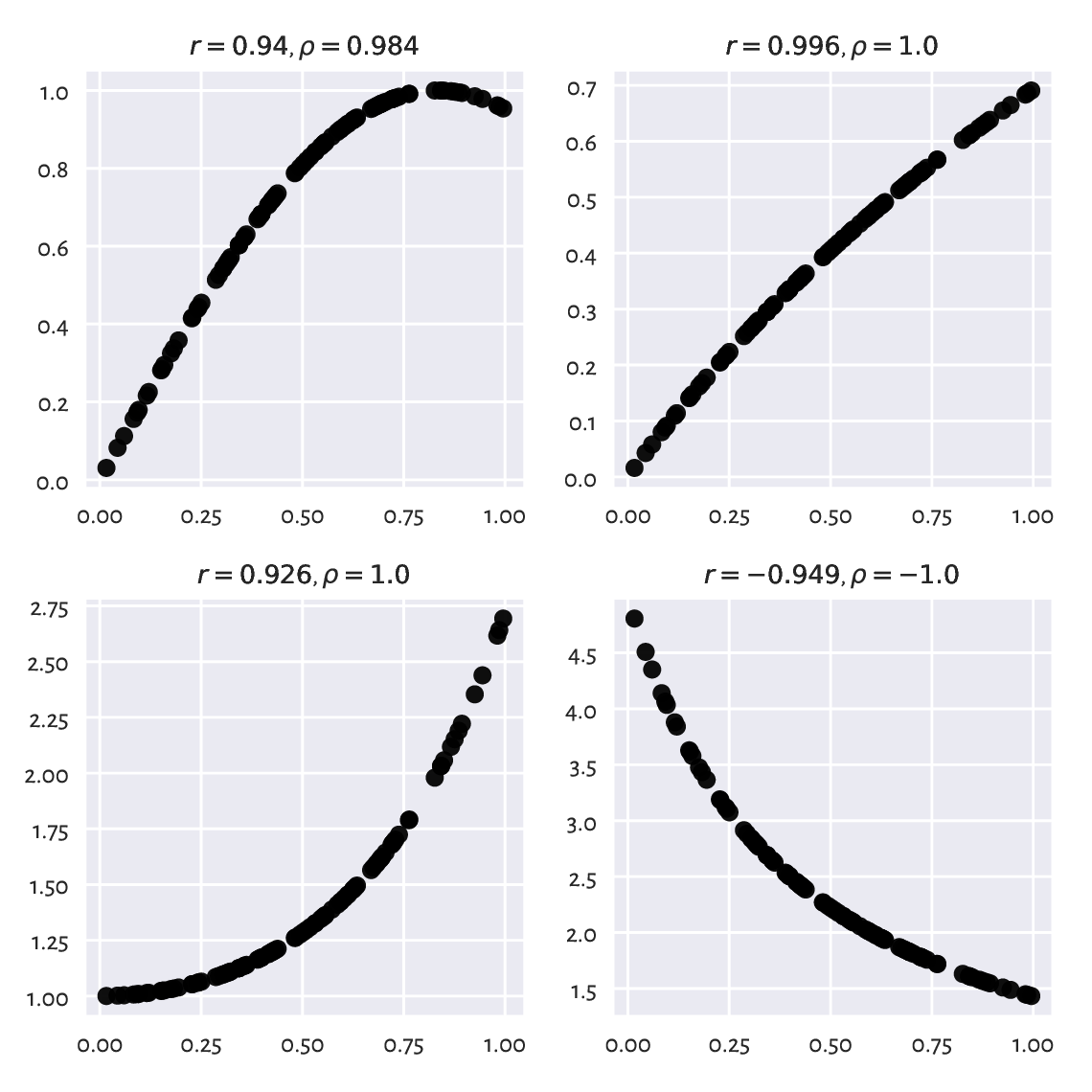Figure 9.4 Example non-linear relationships look like linear to Pearson’s r

No single measure is perfect – we are trying to compress 2n data points into a single number — it is obvious that there will be many different datasets, sometimes very diverse, that will yield the same correlation value.

#### 9.1.1.5. Correlation Is Not Causation

A high correlation degree (either positive or negative) does not mean that there is any casual relationship between the two variables. We cannot say that having large arm circumference affects hip size or the other way around. There might be some latent variable that influences these two (e.g., maybe also related to weight?).

Exercise 9.2

Quite often, medical advice is formulated based on correlations and similar association-measuring tools. We should know how to interpret them, as it is never a true cause-effect relationship; rather, it is all about detecting common patterns in larger populations. For instance, in “obesity increases the likelihood of lower back pain and diabetes” we does not say that one necessarily implies another or that if you are not overweight, there is no risk of getting the two said conditions. It might also work the other way around, as lower back pain may lead to less exercise and then weight gain. Reality is complex. Find similar patterns related to other sets of conditions. Which are stronger than others?

Note

Measuring correlations can aid in constructing regression models, where we would like to identify the transformation that expresses one variable as a function of one or more other ones. When we say that $$y$$ can be modelled by $$ax+b$$, regression analysis will identify some concrete $$a$$ and $$b$$ coefficients – see Section 9.2.3 for more details.

In particular, we would like to include some variables that are correlated with the modelled variable but avoid modelling with the features that are highly correlated with each other, because they do not bring anything interesting to the table and can cause the solution to be numerically unstable.

### 9.1.2. Correlation Heatmap

Calling numpy.corrcoef(body.T) (note the matrix transpose) allows for determining the linear correlation coefficients between all pairs of variables.

We can nicely depict them on a heatmap, see Figure 9.5.

from matplotlib import cm
order = [4, 5, 6, 0, 2, 1, 3]
cols = np.array(["weight", "height", "arm len",
"leg len", "arm circ", "hip circ", "waist circ"])
C = np.corrcoef(body.T)
sns.heatmap(
C[np.ix_(order, order)],
xticklabels=cols[order],
yticklabels=cols[order],
annot=True, fmt=".2f", cmap=cm.get_cmap("copper")
)
plt.show()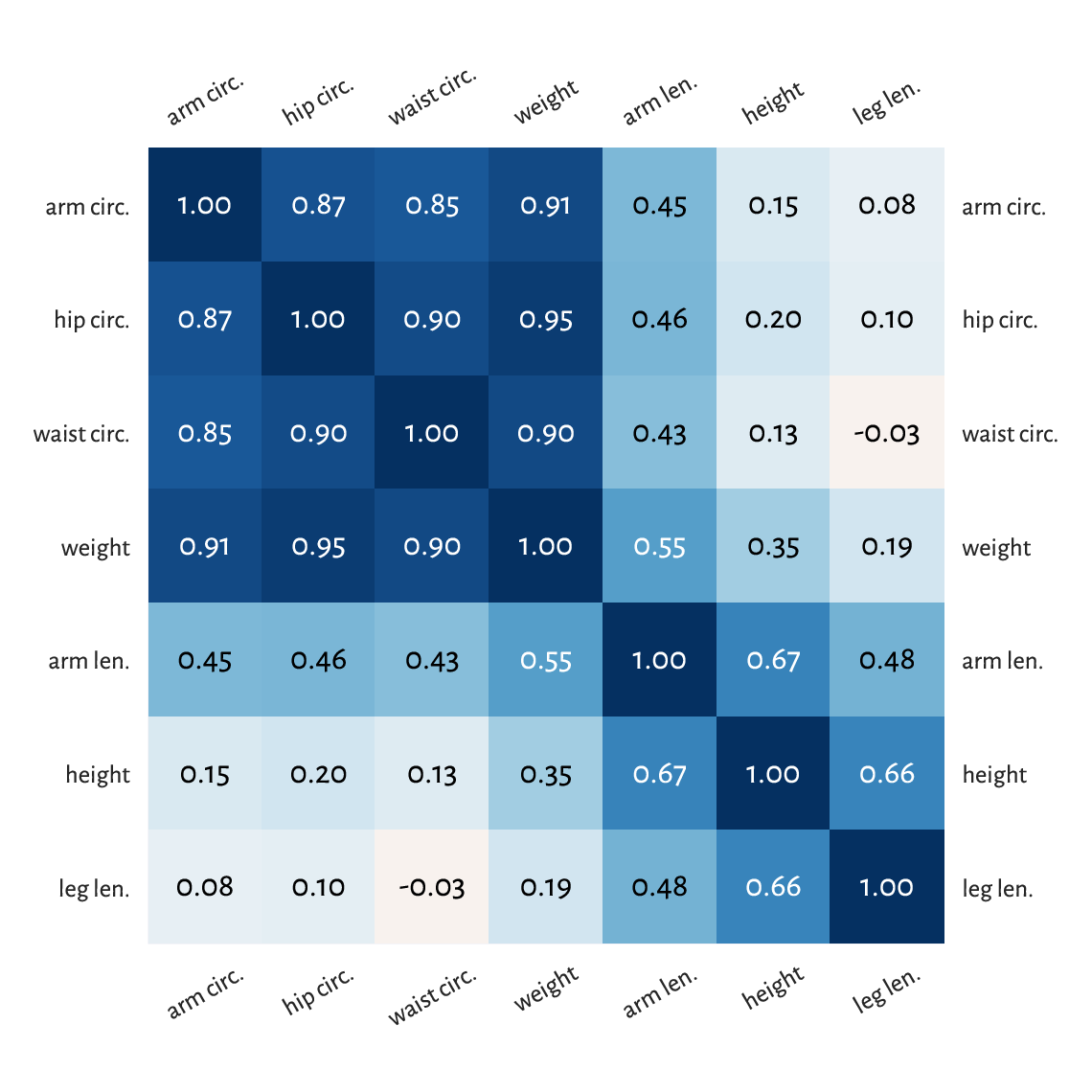Figure 9.5 A correlation heatmap

Notice that we have ordered the columns to reveal some naturally occurring variable clusters: for instance, arm, hip, waist circumference and weight are all quite strongly correlated.

Of course, we have 1.0s on the main diagonal because a variable is trivially correlated with itself. Interestingly, this heatmap is symmetric which is due to the property $$r(\boldsymbol{x}, \boldsymbol{y}) = r(\boldsymbol{y}, \boldsymbol{x})$$.

Example 9.3

(*) To fetch the row and column index of the most correlated pair of variables (either positively or negatively), we should first take the upper (or lower) triangle of the correlation matrix (see numpy.triu or numpy.tril) to ignore the irrelevant and repeating items:

Cu = np.triu(np.abs(C), 1)
np.round(Cu, 2)
## array([[0.  , 0.35, 0.55, 0.19, 0.91, 0.95, 0.9 ],
##        [0.  , 0.  , 0.67, 0.66, 0.15, 0.2 , 0.13],
##        [0.  , 0.  , 0.  , 0.48, 0.45, 0.46, 0.43],
##        [0.  , 0.  , 0.  , 0.  , 0.08, 0.1 , 0.03],
##        [0.  , 0.  , 0.  , 0.  , 0.  , 0.87, 0.85],
##        [0.  , 0.  , 0.  , 0.  , 0.  , 0.  , 0.9 ],
##        [0.  , 0.  , 0.  , 0.  , 0.  , 0.  , 0.  ]])


and then find the location of the maximum:

np.unravel_index(np.argmax(Cu), Cu.shape)
## (0, 5)


Thus, weight and hip circumference is the most strongly correlated.

Note that numpy.argmax returns an index in the flattened (unidimensional) array, therefore we had to use numpy.unravel_index to convert it to a two-dimensional one.

### 9.1.3. Linear Correlation Coefficients on Transformed Data

Pearson’s coefficient can of course also be applied on nonlinearly transformed versions of variables, e.g., logarithms (remember incomes?), squares, square roots, etc.

Let us consider an excerpt from the 2020 CIA World Factbook, where we have data on gross domestic product per capita (based on purchasing power parity) and life expectancy at birth in many countries.

world = pd.read_csv("https://raw.githubusercontent.com/gagolews/" +
"teaching_data/master/marek/world_factbook_2020_subset1.csv",
comment="#")
world = world.to_numpy()
world[:6, :]  # preview
## array([[ 2000. ,    52.8],
##        [12500. ,    79. ],
##        [15200. ,    77.5],
##        [11200. ,    74.8],
##        [49900. ,    83. ],
##        [ 6800. ,    61.3]])


Figure 9.6 depicts these data on a scatterplot.

plt.subplot(1, 2, 1)
plot_corr(world[:, 0], world[:, 1])
plt.xlabel("per capita GDP PPP")
plt.ylabel("life expectancy (years)")
plt.subplot(1, 2, 2)
plot_corr(np.log(world[:, 0]), world[:, 1])
plt.xlabel("log(per capita GDP PPP)")
plt.yticks()
plt.show()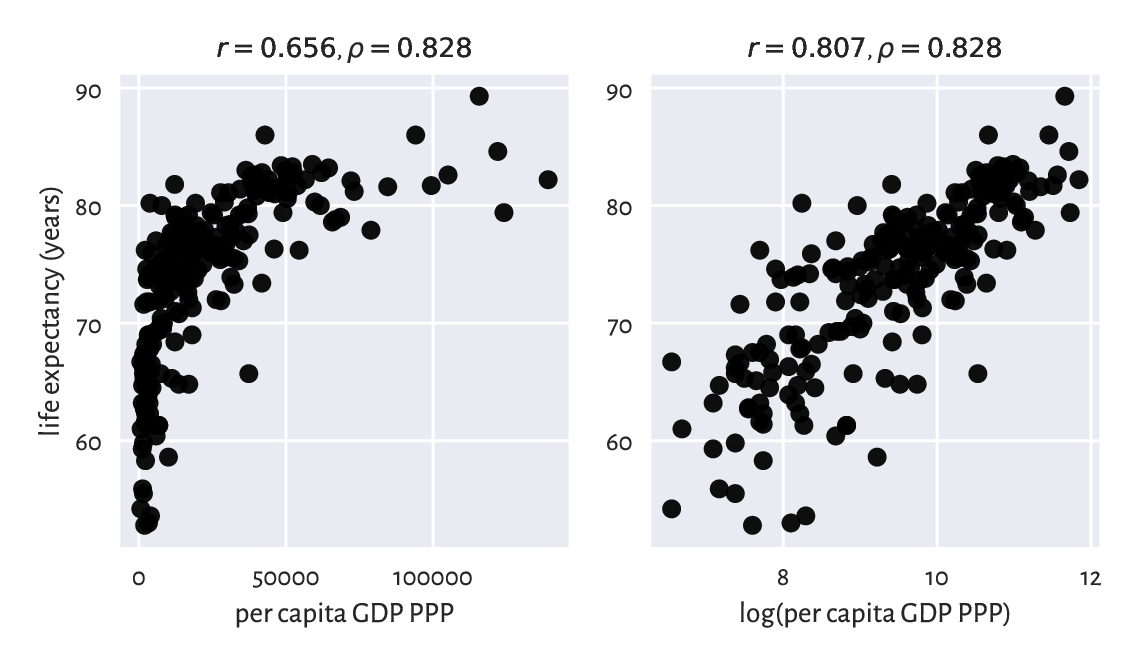Figure 9.6 Scatterplots for life expectancy vs gross domestic product (purchasing power parity) on linear (left-) and log-scale (righthand side)

Computing Pearson’s r between these two indicates a quite weak linear correlation:

scipy.stats.pearsonr(world[:, 0], world[:, 1])
## 0.656471945486374


However, already the logarithm of GDP is slightly more strongly linearly correlated with life expectancy:

scipy.stats.pearsonr(np.log(world[:, 0]), world[:, 1])
## 0.8066505089380016


which means that modelling our data via $$\boldsymbol{y}=a \log\boldsymbol{x}+b$$ can be an idea worth considering.

### 9.1.4. Spearman’s Rank Correlation Coefficient

Sometimes we might be interested in measuring the degree of any kind of monotonic correlation – to what extent one variable is an increasing or decreasing function of another one (linear, logarithmic, quadratic over the positive domain, etc.).

Spearman’s rank correlation coefficient is frequently used in such a scenario:

$\rho(\boldsymbol{x}, \boldsymbol{y}) = r(R(\boldsymbol{x}), R(\boldsymbol{y}))$

which is1 the Pearson coefficient computed over vectors of the corresponding ranks of all the elements in $$\boldsymbol{x}$$ and $$\boldsymbol{y}$$ (denoted with $$R(\boldsymbol{x})$$ and $$R(\boldsymbol{y})$$).

Hence, the two following calls are equivalent:

scipy.stats.spearmanr(world[:, 0], world[:, 1])
## 0.8275220380818622
scipy.stats.pearsonr(
scipy.stats.rankdata(world[:, 0]),
scipy.stats.rankdata(world[:, 1])
)
## 0.8275220380818621


Let us point out that this measure is invariant with respect to monotone transformations of the input variables (up to the sign):

scipy.stats.spearmanr(np.log(world[:, 0]), -np.sqrt(world[:, 1]))
## -0.8275220380818622

Exercise 9.4

We have included the ρs in all the outputs generated by our plot_corr functions. Review all the figures listed above.

Exercise 9.5

Apply numpy.corrcoef and scipy.stats.rankdata (with an appropriate axis argument) to compute the Spearman correlation matrix for all the variable pairs in body. Draw it on a heatmap.

Exercise 9.6

(*) Draw the scatterplots of the ranks of columns in the world and body datasets.

Given a training set of $$n$$ points in an $$m$$-dimensional space represented as an $$\mathbf{X}\in\mathbb{R}^{n\times m}$$ matrix and a set of $$n$$ reference numeric outputs $$\boldsymbol{y}\in\mathbb{R}^n$$, regression aims to find function between the $$m$$ independent/explanatory/predictor variables and a chosen dependent/response/predicted variable:

$y = f(x_1, x_2, \dots, x_m),$

that approximates the given dataset in a usable way.

### 9.2.1. K-Nearest Neighbour Regression

A quite straightforward approach to regression relies on aggregating the reference outputs of the k nearest neighbours of the point tested (compare Section 8.4.4).

For a fixed $$k\ge 1$$ and a given $$\boldsymbol{x}'\in\mathbb{R}^m$$, $$f(\boldsymbol{x}')$$ is computed by averaging the reference outputs in the point’s local neighbourhood.

1. Find the indices $$N_k(\boldsymbol{y})=\{i_1,\dots,i_k\}$$ of the $$k$$ points from $$\mathbf{X}$$ closest to $$\boldsymbol{x}'$$, i.e., ones that fulfil for all $$j\not\in\{i_1,\dots,i_k\}$$

$\|\mathbf{x}_{i_1,\cdot}-\boldsymbol{x}'\| \le\dots\le \| \mathbf{x}_{i_k,\cdot} -\boldsymbol{x}' \| \le \| \mathbf{x}_{j,\cdot} -\boldsymbol{x}' \|.$
2. Return the arithmetic mean of $$(y_{i_1},\dots,y_{i_k})$$ as the result, i.e., assign the average of the outputs corresponding to its $$k$$ nearest neighbours.

Here is a straightforward implementation that generates the predictions for each point in x_test:

def knn_regress(X_test, X_train, y_train, k):
t = scipy.spatial.KDTree(X_train.reshape(-1, 1))
i = t.query(X_test.reshape(-1, 1), k)  # indices of NNs
y = y_train[i]  # corresponding reference outputs
return np.mean(y, axis=1)


For example, let us try expressing weight (the 1st column) as a function of hip circumference (the 6th column) in our NHANES study (body dataset):

$\text{weight}=f_1(\text{hip circumference}) \qquad (+ \text{some error}).$

We will also model the life expectancy at birth in different countries (world dataset) as a function of their GDP per capita (PPP):

$\text{life expectancy}=f_2(\text{GDP per capita}) \qquad (+ \text{some error}).$

Figure 9.7 depicts the fitted functions for a few different ks.

We have obtained a smoothened version of the original dataset. The fact that we do not reproduce the data points in an exact manner is reflected by the (figurative) error term in the above equations. Its role is to emphasise the existence of some natural data variability; after all, one’s weight is not purely determined by their hip size.

For small k we adapt better to the data points, which can be a good thing unless data are very noisy. The greater the k, the smoother the approximation at the cost of losing fine detail and restricted usability at the domain boundary.

Usually, the number of neighbours is chosen by trial and error (just like the number of bins in a histogram; compare Section 4.3.3).

plt.subplot(1, 2, 1)
x = body[:, 5]  # hip circumference
y = body[:, 0]  # weight
plt.plot(x, y, "o", alpha=0.1)
_x = np.linspace(x.min(), x.max(), 1001)
for k in [5, 25, 100]:
_y = knn_regress(_x, x, y, k)
plt.plot(_x, _y, label=f"$k={k}$")
plt.legend()
plt.xlabel("hip circumference")
plt.ylabel("weight")

plt.subplot(1, 2, 2)
x = world[:, 0]  # GDP
y = world[:, 1]  # life expectancy
plt.plot(x, y, "o", alpha=0.1)
_x = np.linspace(x.min(), x.max(), 1001)
for k in [5, 25, 100]:
_y = knn_regress(_x, x, y, k)
plt.plot(_x, _y, label=f"$k={k}$")
plt.legend()
plt.xlabel("per capita GDP PPP")
plt.ylabel("life expectancy (years)")

plt.show()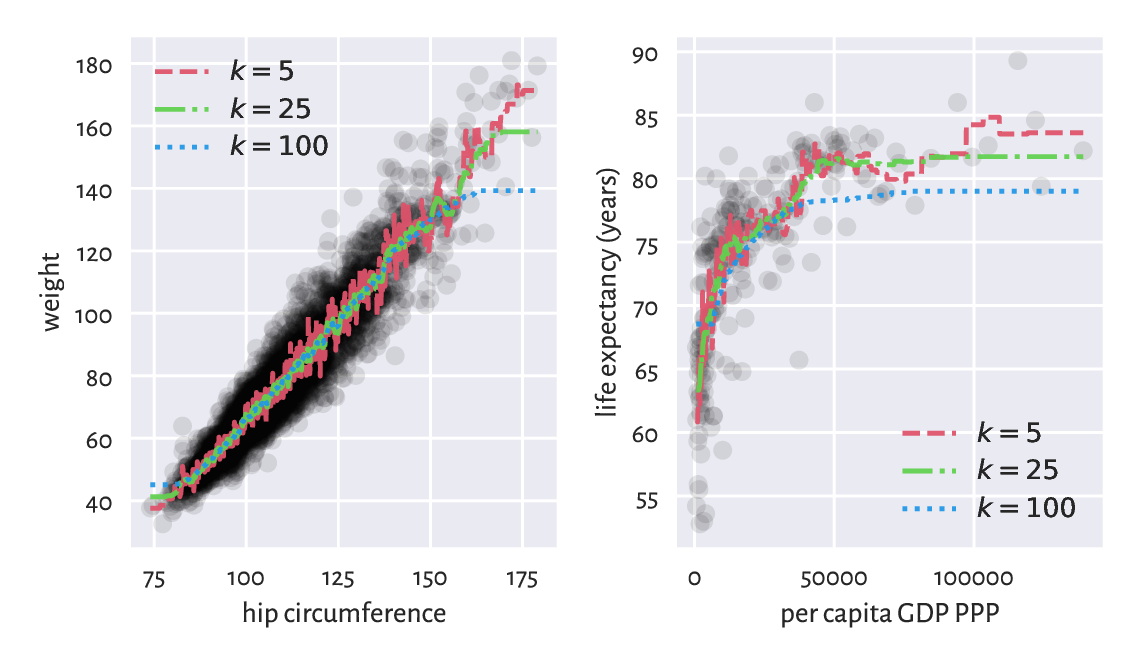Figure 9.7 K-nearest neighbour regression curves for example datasets; the greater the k, the more coarse-grained the approximation

Note

(**) Some methods use weighted arithmetic means for aggregating the k reference outputs, with weights proportional to the actual distances to the neighbours (closer inputs are considered more important).

Also, instead of few nearest neighbours, we could easily implement some form of fixed-radius search regression (compare Section 8.4.4).

These are left as an enjoyable exercise to the skilled reader.

### 9.2.2. From Data to (Linear) Models

Unfortunately, in order to generate predictions for new data points, k-nearest neighbours regression requires that the training sample is available at all times. It does not synthesise or simplify the inputs; instead, it works as a kind of a black-box.

In many contexts we might prefer creating a data model instead, i.e., an easily interpretable mathematical function. A simple yet still quite flexible choice tackles regression problems via linear (affine) maps of the form:

$y = f(x_1, x_2, \dots, x_m) = c_0 + c_1 x_1 + c_2 x_2 + \dots + c_m x_m,$

or, in matrix multiplication terms,

$y = c_0 + \mathbf{c} \mathbf{x}^T,$

where $$\mathbf{c}=[c_1\ c_2\ \cdots\ c_m]$$ and $$\mathbf{x}=[x_1\ x_2\ \cdots\ x_m]$$.

For $$m=1$$, the above simply defines a straight line, which we traditionally denote with

$y = f(x) = ax+b$

i.e., where we mapped $$x \mapsto x_1$$, $$c_0 \mapsto b$$ (intercept), and $$c_1 \mapsto a$$ (slope).

For $$m>1$$, we obtain different hyperplanes (high-dimensional generalisations of the notion of a plane).

Note

As a separate intercept “$$c_0 +$$” term in the defining equation can be quite inconvenient, notationwisely, we usually restrict ourselves to linear maps like

$y = \mathbf{c} \mathbf{x}^T,$

but where we can possibly have an explicit constant $$1$$ component inside $$\mathbf{x}$$, for instance,

$\mathbf{x} = [1\ x_1\ x_2\ \cdots\ x_m].$

Together with $$\mathbf{c} = [c_0\ c_1\ c_2\ \cdots\ c_m]$$, as trivially $$c_0\cdot 1=c_0$$, this new setting is equivalent to the original one.

### 9.2.3. Least Squares Method

A linear model is uniquely2 encoded using only the coefficients $$c_1,\dots,c_m$$. In order to find them, for each point $$\mathbf{x}_{i,\cdot}$$ from the input (training) set, we typically desire the predicted value

$\hat{y}_i = f(x_{i,1}, x_{i,2}, \dots, x_{i,m})= \mathbf{c} \mathbf{x}_{i,\cdot}^T$

to be as close to the corresponding reference $$y_i$$ as possible.

There can be many possible measures of closeness but the most popular one3 uses the notion of the sum of squared residuals (true minus predicted outputs),

$\mathrm{SSR}(\boldsymbol{c}|\mathbf{X},\mathbf{y}) = \sum_{i=1}^n \left( y_i - \hat{y}_i \right)^2 = \sum_{i=1}^n \left( y_i - (c_1 x_{i,1} + c_2 x_{i,2} + \dots + c_m x_{i,m}) \right)^2,$

which is a function of $$\boldsymbol{c}=(c_1,\dots,c_m)$$ (for fixed $$\mathbf{X},\mathbf{y}$$).

And thus the least squares solution to the stated linear regression problem will be defined by the coefficient vector $$\boldsymbol{c}$$ that minimises the SSR. Based on what we have said about matrix multiplication, this is equivalent to solving the optimisation task

$\text{minimise}\ \left(\mathbf{y}-\mathbf{c} \mathbf{X}^T\right) \left(\mathbf{y}-\mathbf{c} \mathbf{X}^T\right)^T \qquad\text{w.r.t. }{(c_1,\dots,c_m)\in\mathbb{R}^m},$

because $$\hat{\mathbf{y}}=\mathbf{c} \mathbf{X}^T$$ gives the predicted values as a row vector (the kind reader is encouraged to check that on a piece of paper now), $$\mathbf{r}=\mathbf{y}-\hat{\mathbf{y}}$$ computes all the $$n$$ residuals, and $$\mathbf{r}\, \mathbf{r}^T$$ gives their sum of squares.

The method of least squares is one of the simplest and most natural approaches to regression analysis (curve fitting). Its theoretical foundations (calculus…) were developed more than 200 years ago by Gauss and then it was polished by Legendre.

Note

(*) Had the points lain on a hyperplane exactly (the interpolation problem), $$\mathbf{y} = \mathbf{c} \mathbf{X}^T$$ would have an exact solution, equivalent to solving the linear system of equations $$\mathbf{y}-\mathbf{c} \mathbf{X}^T =\mathbf{0}$$. However, in our setting we assume that there might be some measurement errors or other discrepancies between the reality and the theoretical model. Thus, to account for this, we are trying to solve a more general problem of finding a hyperplane for which $$\|\mathbf{y}-\mathbf{c} \mathbf{X}^T\|^2$$ is as small as possible.

Note

(*) The above can be solved analytically (compute the partial derivatives of SSR with respect to each $$c_1,\dots,c_m$$, equate them to 0, and solve a simple system of linear equations), which results in $$\mathbf{c} = \mathbf{y} \mathbf{X} (\mathbf{X}^T \mathbf{X})^{-1}$$, where $$\mathbf{A}^{-1}$$ is the inverse of a matrix $$\mathbf{A}$$, i.e., the matrix such that $$\mathbf{A} \mathbf{A}^{-1}=\mathbf{A}^{-1} \mathbf{A} =\mathbf{I}$$. As inverting larger matrices directly is not too robust, numerically speaking, we prefer relying upon some more specialised algorithms to determine the solution.

The scipy.linalg.lstsq function provides a quite numerically stable procedure (which is based on the singular value decomposition of the model matrix, see below for discussion and common pitfalls).

Consider the above NHANES study excerpt one more time; let us say we would like to express weight (the 1st column) as a now linear function of hip circumference (the 6th column),

$\text{weight}=a\cdot\text{hip circumference} + b \qquad (+ \text{some error}).$

The error term corresponds to the residuals as by drawing a scatterplot (see the figure below) of the involved variables we can see that the data do not lie on a straight line perfectly: each model is an idealisation/simplification of the described reality.

The design (model) matrix $$\mathbf{X}$$ and reference $$\boldsymbol{y}$$s are thus:

x_original = body[:, ]
X = x_original**[0, 1]  # 1s, hip circumference
y_true = body[:, 0]  # weight


We have used the vectorised exponentiation operator to convert each $$x_i$$ (the $$i$$-th hip circumference) to a pair $$(x_i^0, x_i^1) = (1, x_i)$$, which is a nice trick to prepended a column of 1s to X so that we can include the intercept term in the model. Here is a preview:

preview_indices = [4, 5, 6, 8, 12, 13]
X[preview_indices, :]
## array([[  1. ,  92.5],
##        [  1. , 106.7],
##        [  1. ,  96.3],
##        [  1. , 102. ],
##        [  1. ,  94.8],
##        [  1. ,  97.5]])
y_true[preview_indices]
## array([55.4, 62. , 66.2, 77.2, 64.2, 56.8])


Let us determine the least squares solution to our regression problem:

import scipy.linalg
res = scipy.linalg.lstsq(X, y_true)


The optimal coefficients vector (one that minimises the SSR) is:

c = res
c
## array([-65.10087248,   1.3052463 ])


Therefore, the estimated model is:

$\text{weight}=1.305\cdot\text{hip circumference} -65.1 \qquad (+ \text{some error}).$

Let us contemplate the fact that the model is nicely interpretable. For instance, with increasing hip circumference we expect the weights be greater. It does not mean that there is some casual relationship between the two (for instance, there can be some latent variables that affect both of them), but rather that there is some general tendency of how the data aligns in the sample space. For instance, that the “best guess” (according to the current model – there can be many, see below) weight for a person with hip circumference of 100 cm is 65.4 kg. Thanks to such models, we can understand certain phenomena better or find some proxies for different variables (especially if measuring them directly is tedious, costly, dangerous, etc.).

Let us determine the predicted weights and display them for the first 6 persons:

y_pred = c @ X.T
np.round(y_pred[preview_indices], 2)
## array([55.63, 74.17, 60.59, 68.03, 58.64, 62.16])


The scatterplot and the fitted regression line in Figure 9.8 indicates a quite good fit, but of course there is some natural variability.

_x = np.array([x_original.min(), x_original.max()]).reshape(-1, 1)
_y = c @ (_x**[0, 1]).T
plt.plot(x_original, y_true, "o", alpha=0.1)
plt.plot(_x, _y, "r-")
plt.xlabel("hip circumference")
plt.ylabel("weight")
plt.show()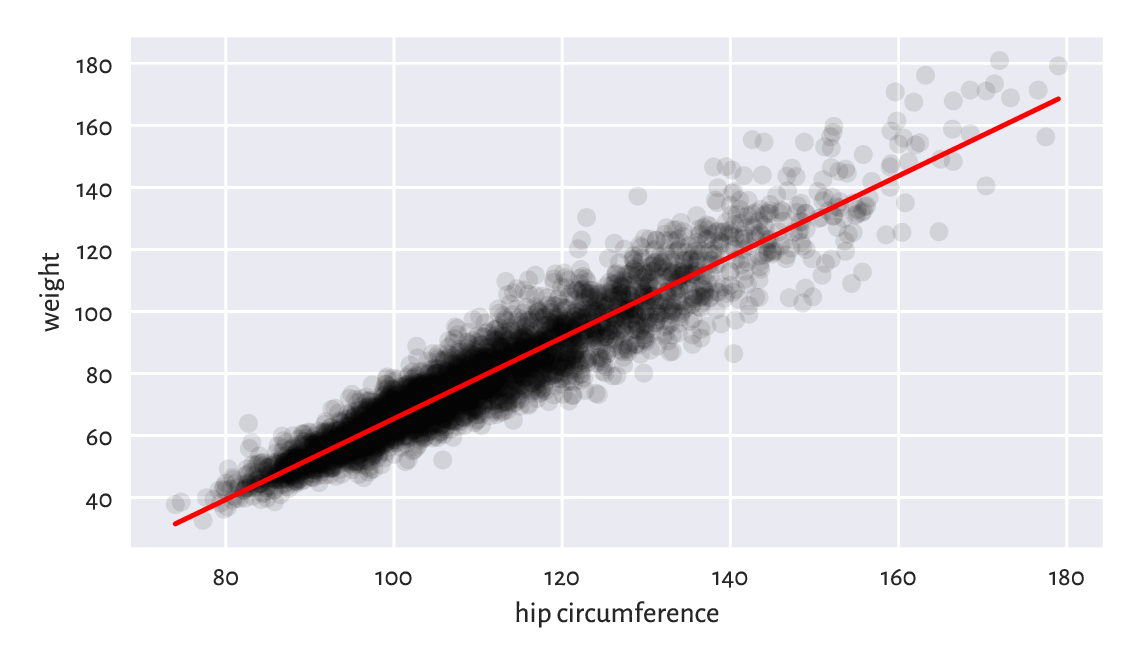Figure 9.8 The least squares line for weight vs hip circumference

Exercise 9.7

The Anscombe quartet is a famous example dataset, where we have 4 pairs of variables having very similar means, variances, linear correlation coefficients, and which can be approximated by the same straight line, however, whose scatter plots are very different. Contemplate upon this toy example yourself.

### 9.2.4. Analysis of Residuals

The residuals, i.e., the estimation errors – what we expected vs what we get, for the chosen 6 observations are:

r = y_true - y_pred
np.round(r[preview_indices], 2)
## array([ -0.23, -12.17,   5.61,   9.17,   5.56,  -5.36])


These residuals are visualised in Figure 9.9.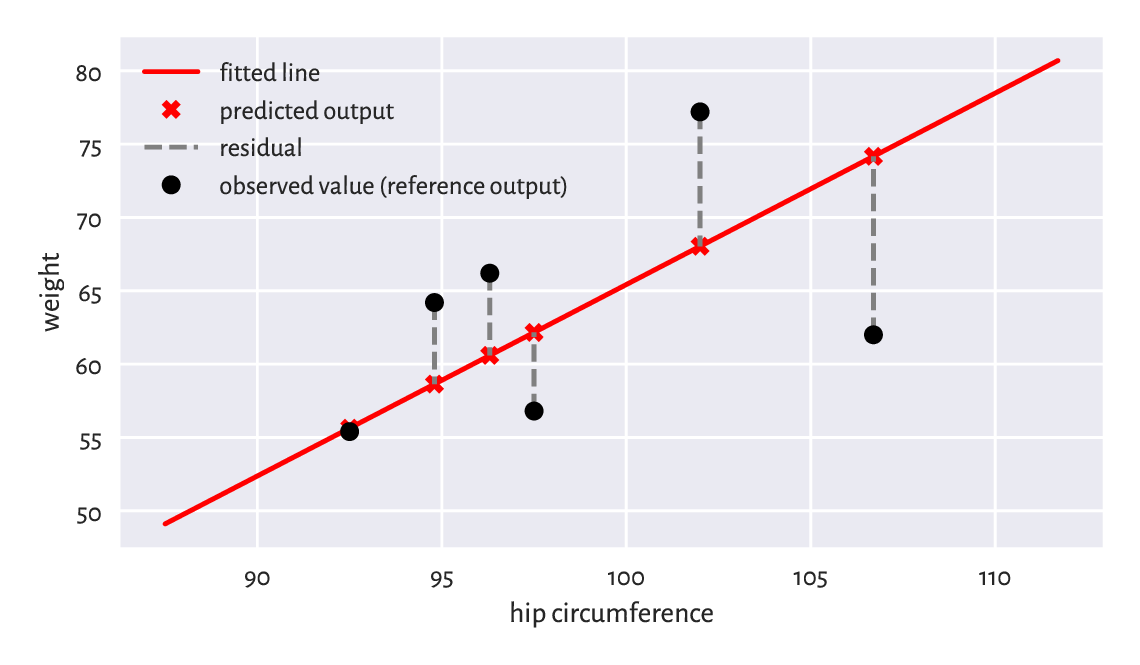Figure 9.9 Example residuals in a simple linear regression task

We wanted the squared residuals (on average – across all the points) be as small as possible, and the least squares method assured that this is the case relative to the chosen model. However, it still does not mean that what we have obtained necessarily constitutes a good fit to the training data. Thus, we need to perform the analysis of residuals.

Interestingly, the average of residuals is always zero:

$\frac{1}{n} \sum_{i=1}^n (y_i - \hat{y}_i) = 0.$

Therefore, if we want to summarise the residuals into a single number, we should rather use, for example, the root mean squared error (RMSE):

$\mathrm{RMSE}(\boldsymbol{c}|\mathbf{X},\mathbf{y}) = \sqrt{\frac{1}{n} \sum_{i=1}^n (y_i-\hat{y}_i)^2}.$
np.sqrt(np.mean(r**2))
## 6.948470091176111


or the mean absolute error:

$\mathrm{MAE}(\boldsymbol{c}|\mathbf{X},\mathbf{y}) = \frac{1}{n} \sum_{i=1}^n |y_i-\hat{y}_i|.$
np.mean(np.abs(r))
## 5.207073583769204


which is nicely interpretable, because it measures by how many kilograms do we err on average. Not bad.

Exercise 9.8

Fit a regression line explaining weight as a function of the waist circumference and compute the corresponding RMSE and MAE.

Note

Generally, fitting simple (involving one independent variable) linear models can only make sense for highly linearly correlated variables. Interestingly, if $$\boldsymbol{y}$$ and $$\boldsymbol{x}$$ are both standardised, and $$r$$ is their Pearson’s coefficient, then the least squares-fitted simple linear model will be given by $$y=rx$$.

In order to verify whether a fitted model is not extremely wrong (e.g., when we fit a liner model to data that clearly follows a different functional relationship), a plot of residuals against the fitted values can be of help; see Figure 9.10. Ideally, the points should be aligned totally at random (homoscedasticity) therein, without any dependence structure.

plt.plot(y_pred, r, "o", alpha=0.1)
plt.axhline(0, ls="--", color="red")
plt.xlabel("fitted values")
plt.ylabel("residuals")
plt.show()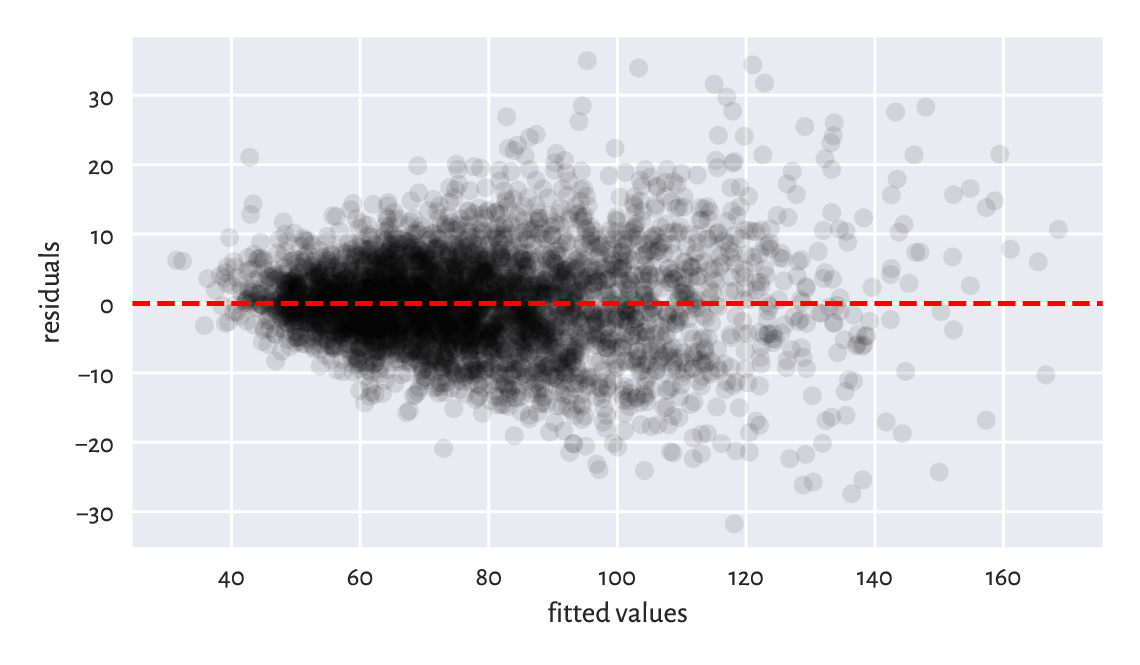Figure 9.10 Residuals vs fitted values for the linear model explaining weight as a function of hip circumference; the variance of residuals slightly increases as $$\hat{y}_i$$ increases, which is not ideal, but it could be much worse than this

Exercise 9.9

Compare4 the RMSE and MAE for the k-nearest neighbour regression curves depicted in the lefthandside of Figure 9.7. Also, draw the residuals vs fitted plot.

For linear models fitted using the least squares method, it can be shown that

$\frac{1}{n} \sum_{i=1}^{n} \left(y_i-\bar{y}\right)^2 = \frac{1}{n} \sum_{i=1}^{n} \left(\hat{y}_i-\bar{\hat{y}}\right)^2 + \frac{1}{n} \sum_{i=1}^{n} \left(y_i-\hat{y}_i\right)^2$

that is, the variance of the dependent variable (left) can be decomposed as the variance of the predicted variables plus the averaged squared residuals. Multiplying the above by $$n$$, we have that the total sum of squares (which we want to be as close to the former as possible) is equal to the explained sum of squares plus the residual sum of squares (which we want to be as small as possible):

$\mathrm{TSS} = \mathrm{ESS} + \mathrm{RSS}.$

The coefficient of determination (unadjusted R-Squared, sometimes referred to as simply the score) is a popular normalised, unitless measure that is quite easy to interpret with no domain-specific knowledge of the modelled problem. Namely, it is given by:

$R^2(\boldsymbol{c}|\mathbf{X},\mathbf{y}) = \frac{\mathrm{ESS}}{\mathrm{TSS}} = 1 - \frac{\mathrm{RSS}}{\mathrm{TSS}} = 1-\frac{s_r^2}{s_y^2},$
1 - np.var(y_true-y_pred)/np.var(y_true)
## 0.8959634726270759


The coefficient of determination in the current context5 is thus the proportion of variance of the dependent variable explained by the independent variables in the model – the closer it is to 1, the better. A dummy model that always returns the mean of $$\boldsymbol{y}$$ gives R-squared of 0.

In our case, $$R^2\simeq 0.9$$ is quite high, which indicates a moderately good fit.

Note

There are certain statistical results that can be relied upon provided that the residuals are independent random variables with expectation zero and the same variance (e.g., the Gauss–Markov theorem). Further, if they are normally distributed, then we have a number of hypothesis tests available (e.g., for the significance of coefficients). This is why various textbooks verify such assumptions. However, we do not go that far in this introductory course.

### 9.2.5. Multiple Regression

As another example, let us fit a model involving two independent variables, arm and hip circumference:

X = np.insert(body[:, [4, 5]], 0, 1, axis=1)  # prepend a column of 1s
res = scipy.linalg.lstsq(X, y_true)
c = res
np.round(c, 2)
## array([-63.38,   1.3 ,   0.9 ])


Thus, we have fitted the plane

$\text{weight} = -63.38 + 1.3\, \text{arm circumference} + 0.9\, \text{hip circumference}.$

We skip the visualisation part, because we do not expect it to result in a readable plot – these are multidimensional data after all. The coefficient of determination is

y_pred = c @ X.T
r = y_true - y_pred
1-np.var(r)/np.var(y_true)
## 0.9243996585518783


Root mean squared error:

np.sqrt(np.mean(r**2))
## 5.923223870044694


Mean absolute error:

np.mean(np.abs(r))
## 4.431548244333894


Thus, it is a slightly better model than the previous one — we can predict the study participants’ weights with better precision, at the cost of increased model complexity.

### 9.2.6. Variable Transformation and Linearisable Models (*)

We are of course not restricted merely to linear functions of the input variables, because by applying arbitrary transformations upon the design matrix columns, we can cover many interesting scenarios.

For instance, a polynomial model involving two variables:

$g(v_1, v_2) = \beta_0 + \beta_1 v_1 + \beta_2 v_1^2 + \beta_3 v_1 v_2 + \beta_4 v_2 + \beta_5 v_2^2$

can be obtained by substituting $$x_1=1$$, $$x_2=v_1$$, $$x_3=v_1^2$$, $$x_4=v_1 v_2$$, $$x_5=v_2$$, $$x_6=v_2^2$$, and then fitting

$f(x_1, x_2, \dots, x_6) = c_1 x_1 + c_2 x_2 + \dots + x_6 x_6.$

The design matrix is made of rubber, it can handle anything (unless it carries highly correlated pairs of input variables). It will still be a linear model, but with respect to transformed data. The algorithm does not care. That is the beauty of the underlying mathematics.

A creative modeller can also turn models such as $$u=c e^{av}$$ into $$y=ax+b$$ by replacing $$y=\log u$$, $$x=v$$, and $$b=\log c$$. There are numerous possibilities here – we call them linearisable models.

As an example, let us model the life expectancy at birth in different countries as a function of their GDP per capita (PPP).

We will consider four different models:

1. $$y=c_1+c_2 x$$ (linear),

2. $$y=c_1+c_2 x+c_3x^2$$ (quadratic),

3. $$y=c_1+c_2 x+c_3x^2+c_4x^4$$ (cubic),

4. $$y=c_1+c_2\log x$$ (logarithmic).

Here are the helper functions that create the model matrices:

def make_model_matrix1(x):
return x.reshape(-1, 1)**[0, 1]

def make_model_matrix2(x):
return x.reshape(-1, 1)**[0, 1, 2]

def make_model_matrix3(x):
return x.reshape(-1, 1)**[0, 1, 2, 3]

def make_model_matrix4(x):
return (np.log(x)).reshape(-1, 1)**[0, 1]

make_model_matrix1.__name__ = "linear model"
make_model_matrix3.__name__ = "cubic model"
make_model_matrix4.__name__ = "logarithmic model"

model_matrix_makers = [
make_model_matrix1,
make_model_matrix2,
make_model_matrix3,
make_model_matrix4
]
Xs = [ make_model_matrix(world[:, 0])
for make_model_matrix in model_matrix_makers ]


Fitting the models:

y_true = world[:, 1]
cs = [ scipy.linalg.lstsq(X, y_true)
for X in Xs ]


Their coefficients of determination are equal to:

for i in range(len(Xs)):
R2 = 1-np.var(y_true - cs[i] @ Xs[i].T)/np.var(y_true)
print(f"{model_matrix_makers[i].__name__:20} R2={R2:.3f}")
## linear model         R2=0.431
## cubic model          R2=0.607
## logarithmic model    R2=0.651


The logarithmic model is thus the best (out of the models we have considered). The four models are depicted in Figure 9.11.

plt.plot(world[:, 0], world[:, 1], "o", alpha=0.1)
_x = np.linspace(world[:, 0].min(), world[:, 0].max(), 101).reshape(-1, 1)
for i in range(len(model_matrix_makers)):
_y = cs[i] @ model_matrix_makers[i](_x).T
plt.plot(_x, _y, label=model_matrix_makers[i].__name__)
plt.legend()
plt.xlabel("per capita GDP PPP")
plt.ylabel("life expectancy (years)")
plt.show()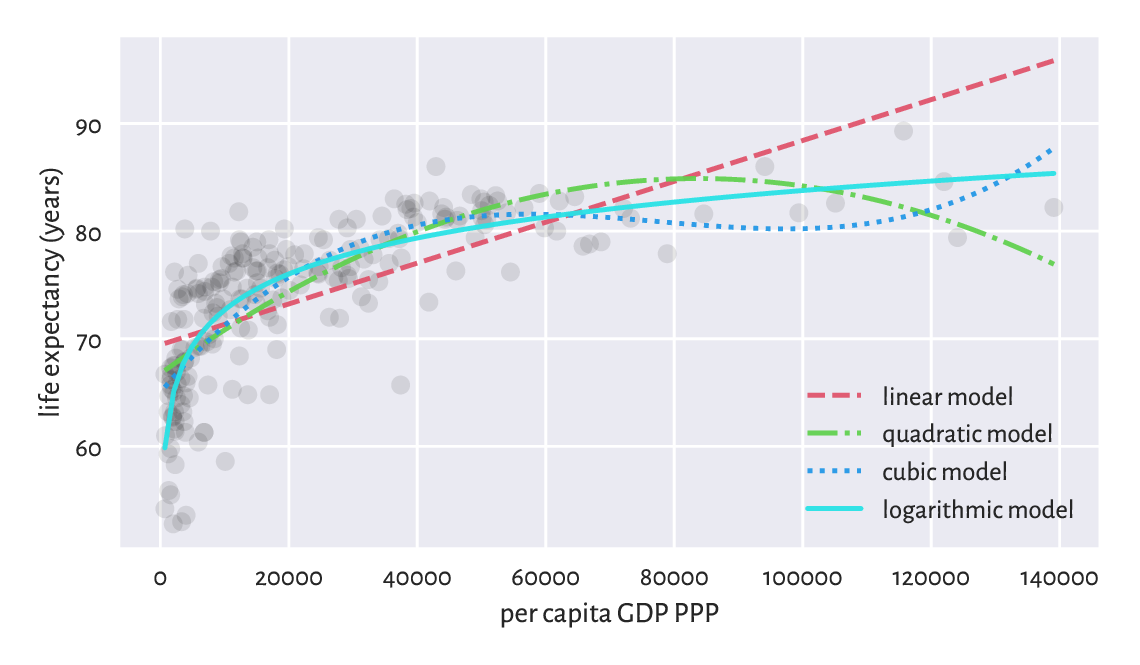Figure 9.11 Different models for life expectancy vs GDP per-capita

Exercise 9.10

Draw box plots and histograms of residuals for each model as well as the scatterplots of residuals vs fitted values.

### 9.2.7. Descriptive vs Predictive Power (*)

We have approximated the life vs GDP relationship using a few different functions. Nevertheless, we see that the above quadratic and cubic models probably do not make much sense, semantically speaking. Sure, they do fit the data better, as far as individual points in the training set are concerned: after all, they have smaller mean squared errors (again: at these given points). However, looking at the way they behave, one does not need a university degree in economics/social policy to conclude that they probably are not the best description of how the reality behaves (on average).

Important

Naturally, a model’s fit to observed data improves as the model’s complexity increases. The Razor principle (by William of Ockham et al.) advises that if some phenomenon can be explained in many different ways, the simplest explanation should be chosen (do not multiply entities [here: introduce independent variables] without necessity).

In particular, the more independent variables we have in the model, the greater the $$R^2$$ coefficient will be. Thus, we can try correcting for this phenomenon by considering the adjusted $$R^2$$

$\bar{R}^2(\boldsymbol{c}|\mathbf{X},\mathbf{y}) = 1 - (1-{R}^2(\boldsymbol{c}|\mathbf{X},\mathbf{y}))\frac{n-1}{n-m-1},$

which, to some extent, penalises more complex models.

Note

(**) Model quality measures adjusted for the number of model parameters, $$m$$, can also be useful in automated variable selection. For example, the Akaike Information Criterion is given by $$\mathrm{AIC}(\boldsymbol{c}|\mathbf{X},\mathbf{y}) = 2m+n\log(\mathrm{SSR}(\boldsymbol{c}|\mathbf{X},\mathbf{y}))-n\log n$$ or the Bayes Information Criterion is defined via $$\mathrm{BIC}(\boldsymbol{c}|\mathbf{X},\mathbf{y}) = m\log n+n\log(\mathrm{SSR}(\boldsymbol{c}|\mathbf{X},\mathbf{y}))-n\log n.$$ However, they are dependent on the scale of $$\boldsymbol{y}$$.

Further, we should also be interested in a model’s predictive power – how well does it generalise to data points that we do not have now (or pretend we do not have), but might face in the future. After all, we observe the modelled reality only at a few different points – the question is how does the model perform when filling the gaps between them.

In particular, we should definitely be careful when extrapolating the model, i.e., making predictions outside of its usual domain. For example, the linear model predicts the following life expectancy for an imaginary country with \$500,000 per capita GDP PPP:

cs @ model_matrix_makers(np.array()).T
## array([164.3593753])


cs @ model_matrix_makers(np.array()).T
## array([-364.10630778])


Nonsense.

Consider the following theoretical example. Let us assume that our true model is $$y=5+3x^3$$.

def true_model(x):
return 5 + 3*(x**3)


Next, we generate a sample from this model but which is – and such is life – subject to some measurement error:

np.random.seed(42)
x = np.random.rand(25)
y = true_model(x) + 0.2*np.random.randn(len(x))


Least-squares fitting of $$y=c_1+c_2 x^3$$ to the above gives:

X = x.reshape(-1, 1)**[0, 3]
c03 = scipy.linalg.lstsq(X, y)
ssr03 = np.sum((y-c03 @ X.T)**2)
np.round(c03, 2)
## array([5.01, 3.13])


which is not very far, but still somewhat distant from the true coefficients, 5 and 3.

However, if we decided to fit a more flexible cubic polynomial, $$y=c_1+c_2 x+c_3 x^2 + c_4 x_3$$

X = x.reshape(-1, 1)**[0, 1, 2, 3]
c0123 = scipy.linalg.lstsq(X, y)
ssr0123 = np.sum((y-c0123 @ X.T)**2)
np.round(c0123, 2)
## array([4.89, 0.32, 0.57, 2.23])


in terms of the SSR, the more complex model is of course better:

ssr03, ssr0123
## (1.0612111154029558, 0.9619488226837543)


but it is farther away from the truth (which, when we perform the fitting task based only on given $$\boldsymbol{x}$$ and $$\boldsymbol{y}$$, is unknown). We may thus say that the first model generalises better on yet-to-be-observed data, see Figure 9.12 for an illustration.

_x = np.linspace(0, 1, 101)
plt.plot(x, y, "o")
plt.plot(_x, true_model(_x), "--", label="true model")
plt.plot(_x, c0123 @ (_x.reshape(-1, 1)**[0, 1, 2, 3]).T,
label="fitted model y=x**[0, 1, 2, 3]")
plt.plot(_x, c03 @ (_x.reshape(-1, 1)**[0, 3]).T,
label="fitted model y=x**[0, 3]")
plt.legend()
plt.show()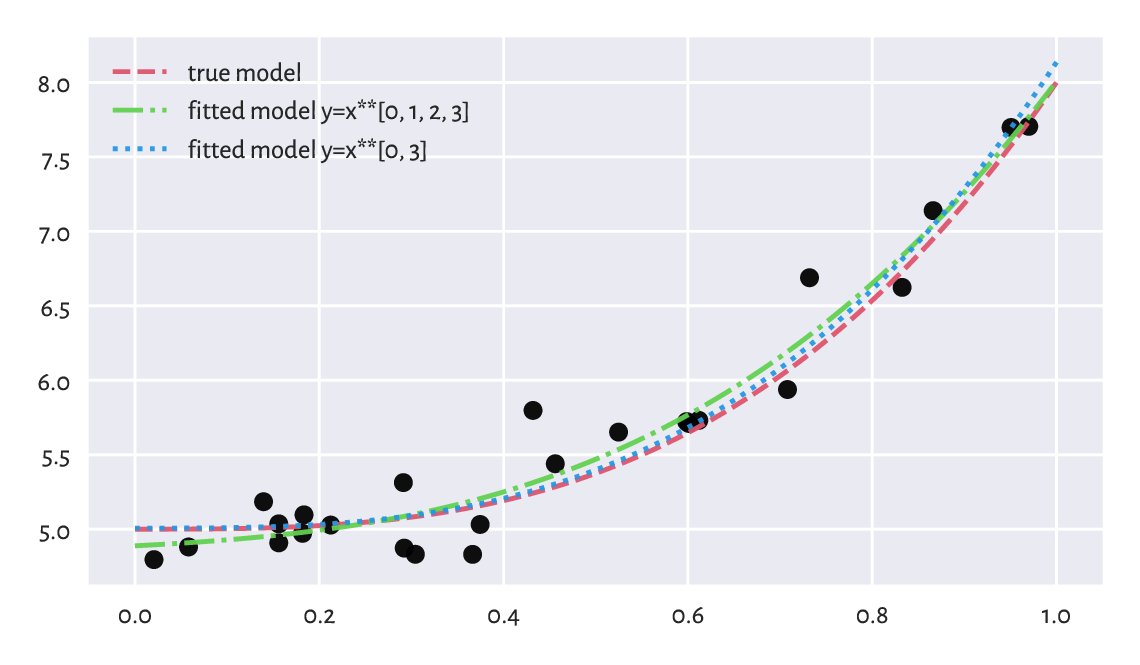Figure 9.12 The true (theoretical) model vs some guesstimates (fitted from noisy data); more degrees of freedom does not necessarily reflect the truth better

We have defined the sum of squared residuals (and its function, the root mean squared error) by means of the averaged deviation from the reference values, which in fact are themselves subject to error. They are our best-shot approximation of the truth, however, they should be taken with a degree of scepticism.

In our case, given the true (reference) model $$f$$ defined over the domain $$D$$ (in our case, $$f(x)=3x^3+5$$ and $$D=[0,1]$$) and an empirically fitted model $$\hat{f}$$, we can replace the sample RMSE with the square root of the integrated squared error:

$\mathrm{RMSE}(\hat{f}|f) = \sqrt{ \int_D (f(x)-\hat{f}(x))^2\, dx }$

which we can approximate numerically by sampling the above at sufficiently many points and applying the trapezoidal rule.

Let us fit a range of polynomial models of different degrees.

ps = np.arange(1, 10)
cs, rmse_train, rmse_test = [], [], []
for p in ps:
c = scipy.linalg.lstsq(x.reshape(-1, 1)**np.arange(p+1), y)
cs.append(c)

y_pred = c @ (x.reshape(-1, 1)**np.arange(p+1)).T
rmse_train.append(np.sqrt(np.mean((y-y_pred)**2)))

_y = c @ (_x.reshape(-1, 1)**np.arange(p+1)).T
_r = (true_model(_x) - _y)**2
rmse_test.append(np.sqrt(0.5*np.sum(
np.diff(_x)*(_r[1:]+_r[:-1])
)))

plt.plot(ps, rmse_train, label="RMSE (training set)")
plt.plot(ps, rmse_test, label="RMSE (theoretical)")
plt.legend()
plt.yscale("log")
plt.xlabel("model complexity (polynomial degree)")
plt.show()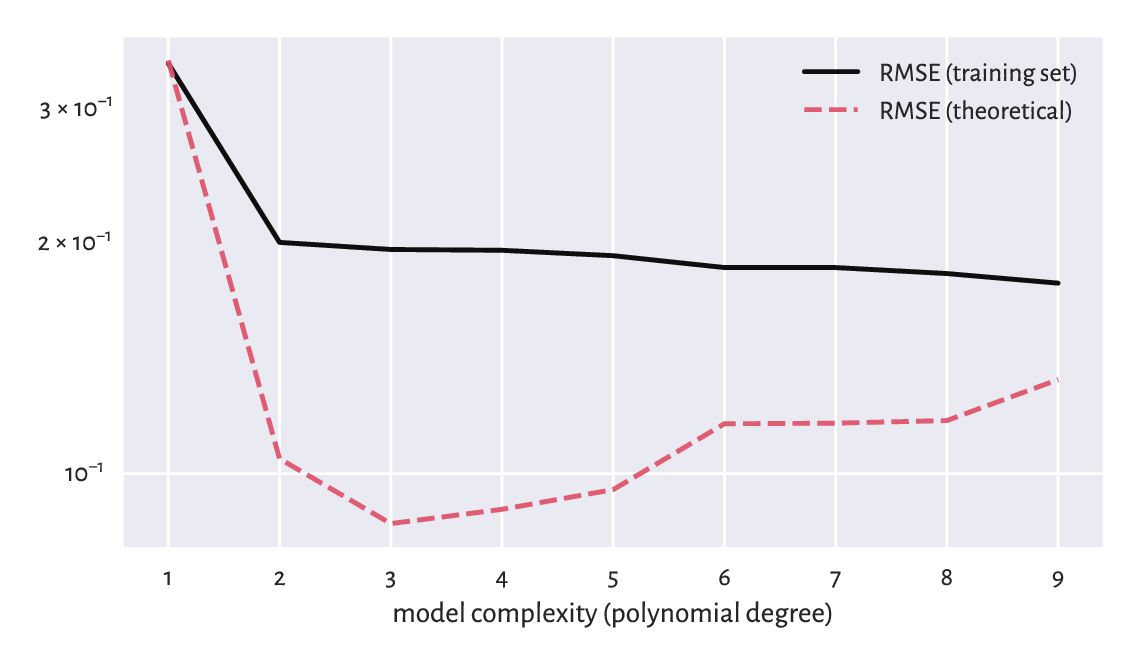Figure 9.13 Good RMSE on training data does not necessarily imply good generalisation abilities

From Figure 9.13 we see that a model’s ability to make correct generalisations to unseen data, with the increased complexity initially improves, but then becomes worse. It is quite a typical behaviour. In fact, the model with the smallest RMSE on the training set, actually overfits to the input sample, see Figure 9.14.

plt.plot(x, y, "o")
plt.plot(_x, true_model(_x), "--", label="true model")
for i in [0, 1, 8]:
plt.plot(_x, cs[i] @ (_x.reshape(-1, 1)**np.arange(ps[i]+1)).T,
label=f"fitted degree-{ps[i]} polynomial")
plt.legend()
plt.show()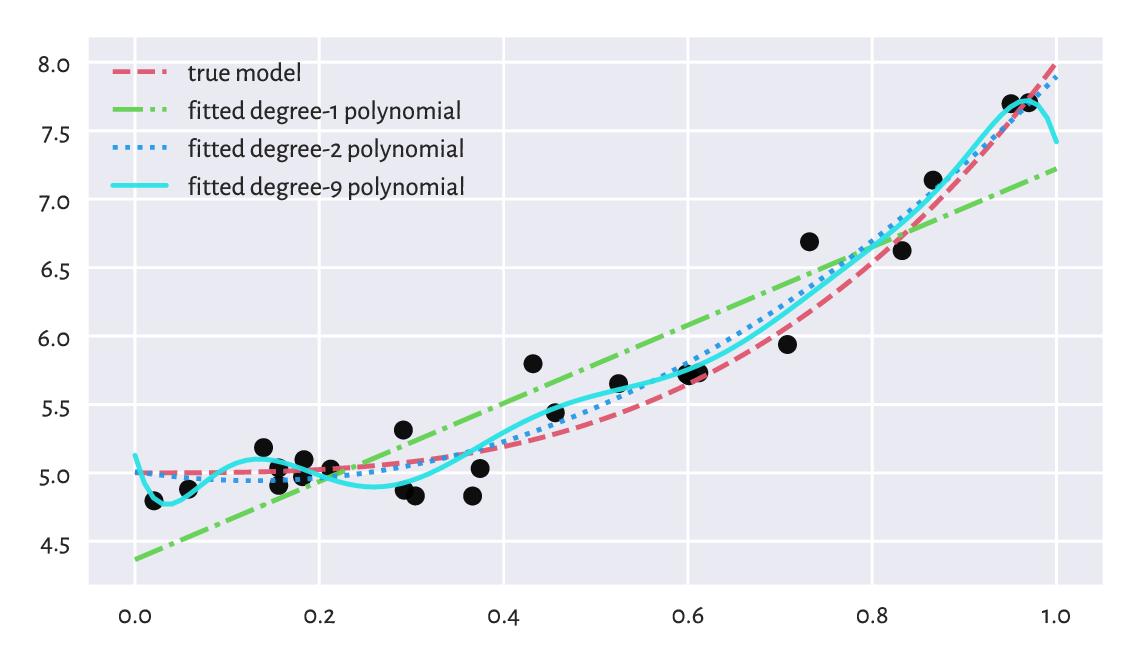Figure 9.14 Under- and overfitting to training data

Important

When evaluating a model’s quality in terms of predictive power on unseen data, we should go beyond inspecting its behaviour merely on the points from the training sample. As the truth is usually not known (if it were, we would not need any guessing), a common approach in case where we have a dataset of a considerable size is to divide it (randomly) into three parts:

• training sample (say, 60%) – used to fit a model,

• test sample (the remaining 40%) – used to assess its quality (e.g., by means of RMSE).

This might emulate an environment where some new data arrives later, see Section 12.3.3 for more details.

Furthermore, if model selection is required, we may apply a training-validation-test split (say, 60/20/20%; see Section 12.3.4) where many models are constructed on the training set, the validation set is used to compute the metrics and choose the best model, and then the test set is employed to come up with the final valuation (because we do not want the model to overfit to the test set).

Overall, models should never be blindly trusted – common sense must always be applied. The fact that we have fitted something using a sophisticated procedure on a dataset that was hard to obtain does not mean we must glorify it. Bad models must be discarded and we should move on. Let us not think about them too much.

### 9.2.8. Fitting Regression Models with scikit-learn (*)

scikit-learn (sklearn; [PVG+11]) is a huge Python package for machine learning built on top of numpy, scipy, and matplotlib. It has a nicely consistent API and implements or provides wrappers for many regression, classification, clustering, and dimensionality reduction algorithms (amongst others).

Important

sklearn is very convenient but allows for fitting models even if we do not understand the mathematics behind them. This is dangerous – it is like driving a manual without a driver’s license only on an autopilot. Advanced students and practitioners will appreciate it, but if used by beginners, it needs to be handled with care; we should not mistake something’s being easily accessible with its being safe to use. Too many bad models go into production and make our daily lives harder. Hence, as a rule, if given a function implementing some procedure and we are not able to provide its definition/mathematical properties/explain its idealised version using pseudocode, we should refrain from using it.

Because of the above, we shall only demo the package’s API. Let us do that by fitting a multiple linear regression model for, again, weight as a function of arm and hip circumference:

X = body[:, [4, 5]]
y_true = body[:, 0]
import sklearn.linear_model
lm = sklearn.linear_model.LinearRegression(fit_intercept=True)
lm.fit(X, y_true)
lm.intercept_, lm.coef_
## LinearRegression()
## (-63.383425410947524, array([1.30457807, 0.8986582 ]))


In scikit-learn, once we construct an object representing the model to be fitted, the fit method will determine the optimal parameters. We have obtained exactly the same solution – it is the same method, after all.

Computing the predicted values can be done by means of the predict method. For example, we can calculate the coefficient of determination:

y_pred = lm.predict(X)
import sklearn.metrics
sklearn.metrics.r2_score(y_true, y_pred)
## 0.9243996585518783


This function is convenient, but do we remember the formula for the score? We should always do.

### 9.2.9. Ill-Conditioned Model Matrices (*)

Our approach to regression analysis relies on solving an optimisation problem (the method least squares). However, sometimes the “optimal” solution that the algorithm returns might have nothing to do with the true minimum. And this is despite the fact that we have the theoretical results (the objective is convex) stating that the solution is unique (there are methods in statistical learning where there might be multiple local minima – this is even more difficult; see Section 12.4.4). The problem stems from our using the computer’s finite-precision floating point arithmetic.

Let us fit a degree-4 polynomial to the life expectancy vs per capita GDP dataset.

X = world[:, ]**[0,1,2,3,4]
y_true = world[:, 1]
cs = dict()


We store the estimated model coefficients in a dictionary, because many methods will follow next. First, scipy:

res = scipy.linalg.lstsq(X, y_true)
cs["scipy_X"] = res
cs["scipy_X"]
## array([ 2.33103950e-16,  6.31514064e-12,  1.34162021e-07,
##        -2.33980973e-12,  1.03490968e-17])


If we drew the fitted polynomial (see the figure below), we would see that the fit is actually very very bad. The result returned by lstsq in this case is not at all optimal. It turns out that the fitting problem is very ill-conditioned (and it is not the algorithm’s fault): GDPs range from very small to very large ones, and taking the powers of 4 thereof results in numbers of ever greater range. Finding the least squares solution involves some matrix inverse (not necessarily directly) and such a matrix might be close to singular.

As a measure of the model matrix’s ill-conditioning, we often use the so-called condition number, being the ratio of the largest to the smallest so-called singular values6 of $$\mathbf{X}^T$$, which are in fact returned by the lstsq method:

s = res
s / s[-1]
## 9.101246653543121e+19


As a rule of thumb, if the condition number is $$10^k$$, we are losing $$k$$ digits of numerical precision when performing the underlying computations. This is thus a very ill-conditioned problem, because the above number is very large.

Note

(**) The least squares regression problem can be solved by means of the singular value decomposition of the model matrix, see Section 9.3.4. Let $$\mathbf{U}\mathbf{S}\mathbf{Q}$$ be the SVD of $$\mathbf{X}^T$$. Then $$\mathbf{c} = \mathbf{U} (1/\mathbf{S}) \mathbf{Q} \mathbf{y}$$, with $$(1/\mathbf{S})=\mathrm{diag}(1/s_{1,1},\dots,1/s_{m,m})$$. Interestingly, as $$s_{1,1}\ge\dots\ge s_{m,m}$$ gives the singular values of $$\mathbf{X}^T$$ (square roots of the eigenvalue of $$\mathbf{X}^T \mathbf{X}$$), the aforementioned condition number can simply be computed as $$s_{1,1}/s_{m,m}$$.

Let us verify the approach used by scikit-learn, which fits the intercept separately (but still, it is merely a wrapper around lstsq with a different API), and so should be slightly better-behaving:

import sklearn.linear_model
lm = sklearn.linear_model.LinearRegression(fit_intercept=True)
lm.fit(X[:, 1:], y_true)
cs["sklearn"] = np.r_[lm.intercept_, lm.coef_]
cs["sklearn"]
## LinearRegression()
## array([ 6.92257641e+01,  5.05754568e-13,  1.38835855e-08,
##        -2.18869526e-13,  9.09347414e-19])


Here is the condition number:

lm.singular_ / lm.singular_[-1]
## 1.4025984282521556e+16


The condition number is also huge – and scikit-learn did not warn us about it. Had we trusted the solution returned by it, we would end up with conclusions from our data analysis built on sand.

If the model matrix is almost singular, the computation of its inverse is prone to enormous numerical errors. One way of dealing with this is to remove highly correlated variables (the multicollinearity problem).

Interestingly, standardisation can sometimes make the fitting more numerically stable. Let $$\mathbf{Z}$$ be a standardised version of the model matrix $$\mathbf{X}$$ with the intercept part (the column of 1s) not included, i.e., with $$\mathbf{z}_{\cdot,j} = (\mathbf{x}_{\cdot,j}-\bar{x}_j)/s_j$$ with $$\bar{x}_j$$ and $$s_j$$ denoting the arithmetic mean and standard deviation of the j-th column in $$\mathbf{X}$$. If $$(d_1,\dots,d_{m-1})$$ is the least squares solution for $$\mathbf{Z}$$, then the least squares solution to the underlying original regression problem is

$\left( \bar{y}-\sum_{j=1}^{m-1} \frac{d_j}{s_j} \bar{x}_j, \frac{d_1}{s_1}, \frac{d_2}{s_2}, \dots, \frac{d_{m-1}}{s_{m-1}} \right)$

with the first term corresponding to the intercept.

Let us test this approach with scipy.linalg.lstsq.

means = np.mean(X[:, 1:], axis=0)
stds = np.std(X[:, 1:], axis=0)
Z = (X[:, 1:]-means)/stds
resZ = scipy.linalg.lstsq(Z, y_true)
c_scipyZ = resZ/stds
cs["scipy_Z"] = np.r_[np.mean(y_true) - (c_scipyZ @ means.T), c_scipyZ]
cs["scipy_Z"]
## array([ 6.35946784e+01,  1.04541932e-03, -2.41992445e-08,
##         2.39133533e-13, -8.13307828e-19])


The condition number is:

s = resZ
s / s[-1]
## 139.4279225737235


This is still not too good (we would prefer a value close to 1) but nevertheless way better.

Figure 9.15 depicts the three fitted models, each claiming to be the solution to the original regression problem.

plt.plot(world[:, 0], world[:, 1], "o", alpha=0.1)
_x = np.linspace(world[:, 0].min(), world[:, 0].max(), 101).reshape(-1, 1)
_X = _x**[0,1,2,3,4]
for lab, c in cs.items():
ssr = np.sum((y_true-c @ X.T)**2)
plt.plot(_x, c @ _X.T, label=f"{lab:10} SSR={ssr:.2f}")
plt.legend()
plt.ylim(20, 120)
plt.xlabel("per capita GDP PPP")
plt.ylabel("life expectancy (years)")
plt.show()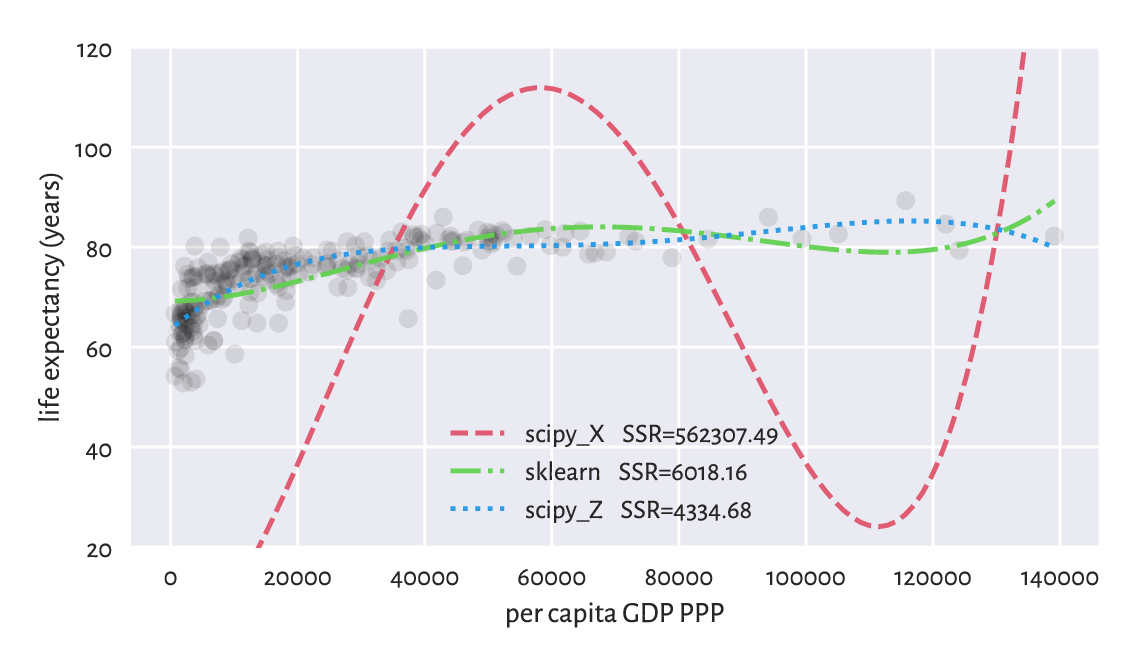Figure 9.15 Ill-conditioned model matrix can result in the resulting models be very wrong

Important

Always check the model matrix’s condition number.

To be strict, if we read a paper in, say, social or medical sciences (amongst others) where the researchers fit a regression model but do not provide the model matrix’s condition number, we should doubt the conclusions they make.

On a final note, we might wonder why the standardisation is not done automatically by the least squares solver. As usual with most numerical methods, there is no one-fits-all solution: e.g., when there are columns of very small variance or there are outliers in data. This is why we need to study all the topics deeply: to be able to respond flexibly to many different scenarios ourselves.

## 9.3. Finding Interesting Combinations of Variables (*)

### 9.3.1. Dot Products, Angles, Collinearity, and Orthogonality

It turns out that the dot product (Section 8.3) has a nice geometrical interpretation:

$\boldsymbol{x}\cdot\boldsymbol{y} = \|\boldsymbol{x}\|\, \|\boldsymbol{y}\|\, \cos\alpha,$

where $$\alpha$$ is the angle between the two vectors. In other words, it is the product of the lengths of the two vectors and the cosine of the angle between them.

We can obtain the cosine part by computing the dot product of the normalised vectors, i.e., such that their lengths are equal to 1:

$\cos\alpha = \frac{\boldsymbol{x}}{\|\boldsymbol{x}\|}\cdot\frac{\boldsymbol{y}}{\|\boldsymbol{y}\|}.$

For example, consider two vectors in $$\mathbb{R}^2$$, $$(1/2, 0)$$ and $$(\sqrt{2}/2, \sqrt{2}/2)$$, which are depicted in Figure 9.16.

u = np.array([0.5, 0])
v = np.array([np.sqrt(2)/2, np.sqrt(2)/2])


Their dot product is equal to:

np.sum(u*v)
## 0.3535533905932738


The dot product of their normalised versions, i.e., the cosine of the angle between them is:

u_norm = u/np.sqrt(np.sum(u*u))
v_norm = v/np.sqrt(np.sum(v*v))  # BTW: this vector was already normalised
np.sum(u_norm*v_norm)
## 0.7071067811865476


The angle itself can be determined by referring to the inverse of the cosine function, i.e., arccosine.

np.arccos(np.sum(u_norm*v_norm)) * 180/np.pi
## 45.0


Notice that we have converted the angle from radians to degrees.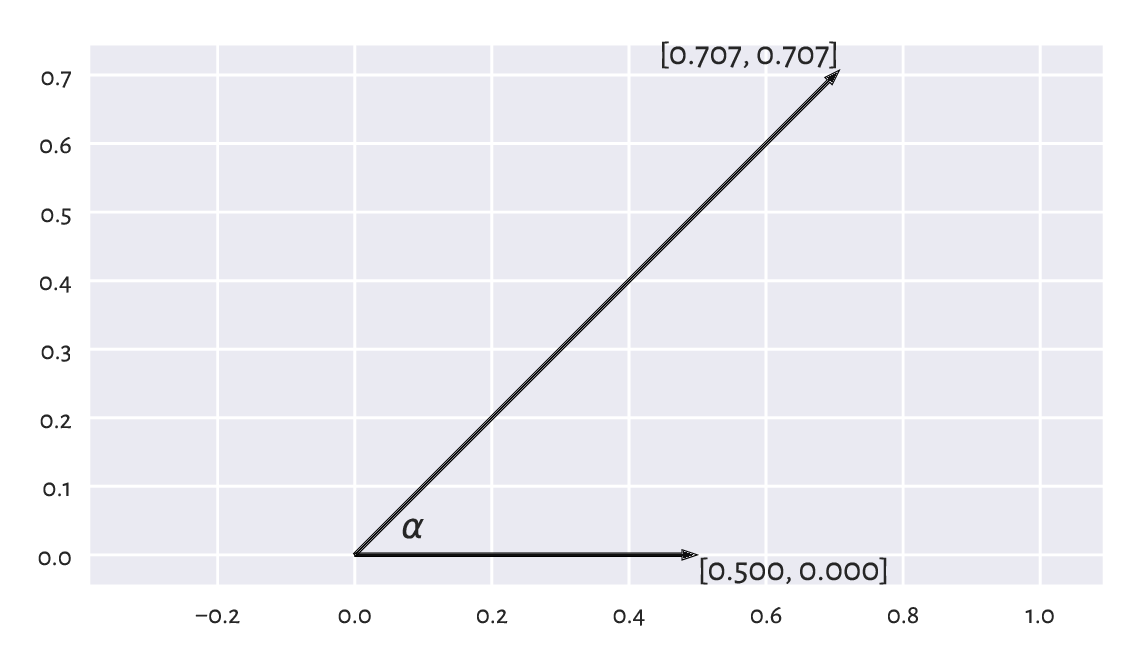Figure 9.16 Example vectors and the angle between them

Important

If two vectors are collinear (codirectional, one is a scaled version of another, angle 0), then $$\cos 0 = 1$$. If they point to opposite directions (-180 degrees angle), then $$\cos \pi=-1$$. For orthogonal (perpendicular, 90 or -90 degree angle) vectors, we get $$\cos(\pi/2)=\cos(-\pi/2)=0$$.

Note

(**) The standard deviation $$s$$ of a vector $$\boldsymbol{x}$$ that has already been centred (whose mean is 0) is a scaled version of the Euclidean norm (divided by the square root of the number of elements, $$n$$), i.e., $$s = \|\boldsymbol{x}\|/\sqrt{n}$$. Looking at the definition of the Pearson linear correlation coefficient, we see that it is the dot product of the standardised versions of two vectors $$\boldsymbol{x}$$ and $$\boldsymbol{y}$$ divided by the number of elements therein. However, if the vectors are centred, we can rewrite the formula equivalently as $$r(\boldsymbol{x}, \boldsymbol{y})= \frac{\boldsymbol{x}}{\|\boldsymbol{x}\|}\cdot\frac{\boldsymbol{y}}{\|\boldsymbol{y}\|}= \cos\alpha$$. It is not easy to imagine vectors in very high dimensional spaces, but at least the fact that $$r$$ is bounded by -1 and 1 is implied from this observation.

### 9.3.2. Geometric Transformations of Points

For certain square matrices of size m by m, matrix multiplication can be thought of as an application of the corresponding geometrical transformation.

Let $$\mathbf{X}$$ be a matrix of shape n by m, which we treat as representing the coordinates of n points in an m-dimensional space. For instance, if we are given a diagonal matrix:

$\begin{split} \mathbf{S}=\mathrm{diag}(s_1, s_2,\dots, s_m)= \left[ \begin{array}{cccc} s_1 & 0 & \dots & 0 \\ 0 & s_2 & \dots & 0 \\ \vdots & \vdots & \ddots & \vdots \\ 0 & 0 & \dots & s_m \\ \end{array} \right], \end{split}$

then $$\mathbf{X} \mathbf{S}$$ represents scaling (stretching) with respect to the axes of the coordinate system in use, because:

$\begin{split} \mathbf{X} \mathbf{S} = \left[ \begin{array}{cccc} s_1 x_{1,1} & s_2 x_{1,2} & \dots & s_m x_{1,m} \\ s_1 x_{2,1} & s_2 x_{2,2} & \dots & s_m x_{2,m} \\ \vdots & \vdots & \ddots & \vdots \\ s_1 x_{n-1,1} & s_2 x_{n-1,2} & \dots & s_m x_{n-1,m} \\ s_1 x_{n,1} & s_2 x_{n,2} & \dots & s_m x_{n,m} \\ \end{array} \right]. \end{split}$

In numpy this can be implemented without actually referring to matrix multiplication; a notation like X * np.array([s1, s2, ..., sm]).reshape(1, -1) will suffice (elementwise multiplication and proper shape broadcasting).

Furthermore, if $$\mathbf{Q}$$ is an orthonormal matrix, i.e., a square matrix whose columns and rows are unit vectors (normalised), all orthogonal to each other, then $$\mathbf{X}\mathbf{Q}$$ represents a combination of rotations and reflections.

Orthonormal matrices are sometimes simply referred to as orthogonal ones.

Important

By definition, a matrix $$\mathbf{Q}$$ is orthonormal if and only if $$\mathbf{Q}^T \mathbf{Q} = \mathbf{Q} \mathbf{Q}^T = \mathbf{I}$$. It is due to the $$\cos(\pi/2) = \cos(-\pi/2)=0$$ interpretation of the dot products of normalised orthogonal vectors.

In particular, for any angle $$\alpha$$, the matrix representing rotations in $$\mathbb{R}^2$$,

$\begin{split} \mathbf{R}(\alpha) = \left[ \begin{array}{cc} \cos \alpha & \sin\alpha \\ -\sin \alpha & \cos\alpha \\ \end{array} \right], \end{split}$

is orthonormal (which can be easily verified using the basic trigonometric equalities).

Furthermore,

$\begin{split} \left[ \begin{array}{cc} 1 & 0 \\ 0 & -1 \\ \end{array} \right] \quad\text{ and }\quad \left[ \begin{array}{cc} -1 & 0 \\ 0 & 1 \\ \end{array} \right] \end{split}$

represent the two reflections, one against the x- and the other against the y-axis, respectively. Both are orthonormal matrices as well.

Consider a dataset $$\mathbf{X}$$ in $$\mathbb{R}^2$$:

np.random.seed(12345)
X = np.random.randn(10000, 2) * 0.25


and its scaled, rotated, and translated (shifted) version

$\begin{split} \mathbf{Y} = \mathbf{X}\ \left[ \begin{array}{cc} 2 & 0 \\ 0 & 0.5 \\ \end{array} \right]\ \left[ \begin{array}{cc} \cos \frac{\pi}{6} & \sin\frac{\pi}{6} \\ -\sin \frac{\pi}{6} & \cos\frac{\pi}{6} \\ \end{array} \right] + \left[ \begin{array}{cc} 3 & 2 \\ \end{array} \right]. \end{split}$
t = np.array([3, 2])
S = np.diag([2, 0.5])
S
## array([[2. , 0. ],
##        [0. , 0.5]])
alpha = np.pi/6
Q = np.array([
[ np.cos(alpha), np.sin(alpha)],
[-np.sin(alpha), np.cos(alpha)]
])
Q
## array([[ 0.8660254,  0.5      ],
##        [-0.5      ,  0.8660254]])
Y = X @ S @ Q + t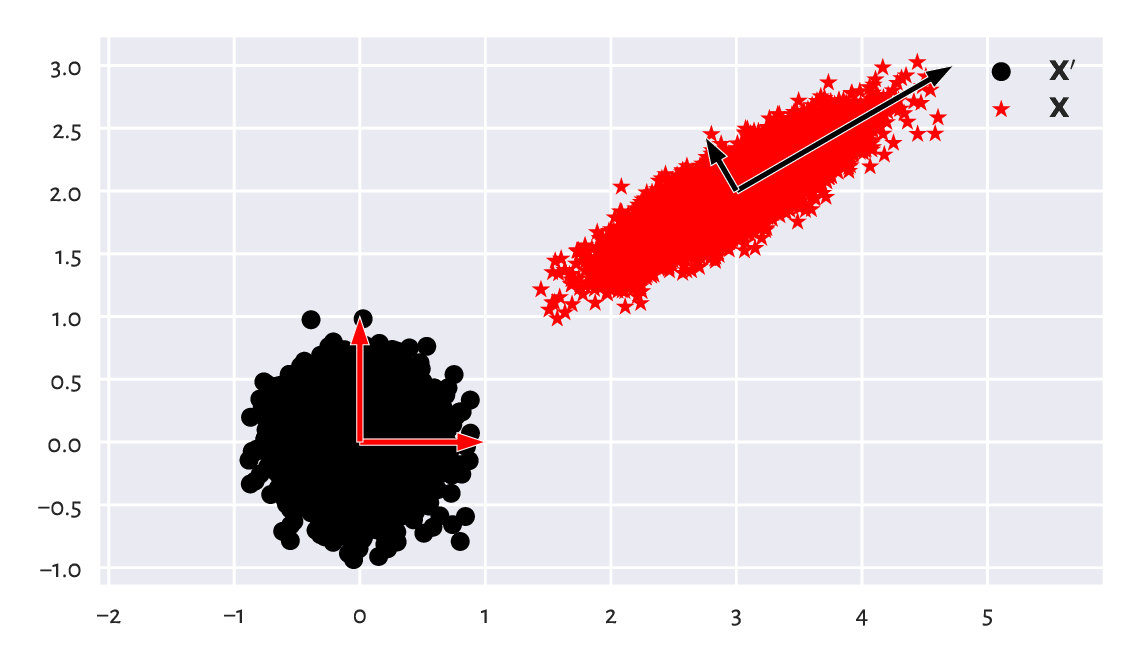Figure 9.17 A dataset and its scaled, rotated, and shifted version

We can consider $$\mathbf{Y}=\mathbf{X} \mathbf{S} \mathbf{Q} + \mathbf{t}$$ a version of $$\mathbf{X}$$ in a new coordinate system (basis), see Figure 9.17. Each column in the transformed matrix is a shifted linear combination of the columns in the original matrix:

$\mathbf{y}_{\cdot,j} = t_j + \sum_{k=1}^m (s_{k, k} q_{k, j}) \mathbf{x}_{\cdot, k}.$

Computing such linear combinations of columns is natural during a dataset’s preprocessing step, especially if they are on the same scale or they are unitless. Actually, the standardisation itself is a form of scaling and translation.

Exercise 9.11

Assume that we have a dataset with two columns, giving the number of apples and the number of oranges in a fresh veggie market clients’ baskets. What kind of orthonormal and scaling transforms should be applied to obtain a matrix bearing the total number of fruits and surplus apples (e.g., a row $$(4, 7)$$ should be converted to $$(11, -3)$$)?

### 9.3.3. Matrix Inverse

The inverse of a square matrix $$\mathbf{A}$$ (if it exists) is denoted with $$\mathbf{A}^{-1}$$ – it is the matrix fulfilling the identity

$\mathbf{A}^{-1} \mathbf{A} = \mathbf{A} \mathbf{A}^{-1} = \mathbf{I}.$

Noting that the identity matrix $$\mathbf{I}$$ is the neutral element of the matrix multiplication, the above is thus the analogue of the inverse of a scalar: something like $$3 \cdot 3^{-1} = 3\cdot \frac{1}{3} = \frac{1}{3} \cdot 3 = 1$$.

Important

For any invertible matrices of admissible shapes, it might be shown that the following noteworthy properties hold:

• $$(\mathbf{A}^{-1})^T = (\mathbf{A}^T)^{-1}$$,

• $$(\mathbf{A}\mathbf{B})^{-1} = \mathbf{B}^{-1} \mathbf{A}^{-1}$$,

• a matrix equality $$\mathbf{A}=\mathbf{B}\mathbf{C}$$ holds if and only if $$\mathbf{A} \mathbf{C}^{-1}=\mathbf{B}\mathbf{C}\mathbf{C}^{-1}=\mathbf{B}$$; this is also equivalent to $$\mathbf{B}^{-1} \mathbf{A}=\mathbf{B}^{-1} \mathbf{B}\mathbf{C}=\mathbf{C}$$.

Matrix inverse allows us to identify the inverses of geometrical transformations. Knowing that $$\mathbf{Y} = \mathbf{X}\mathbf{S}\mathbf{Q}+\mathbf{t}$$, we can recreate the original matrix by applying:

$\mathbf{X} = (\mathbf{Y}-\mathbf{t}) (\mathbf{S}\mathbf{Q})^{-1} = (\mathbf{Y}-\mathbf{t}) \mathbf{Q}^{-1} \mathbf{S}^{-1}.$

It is worth knowing that if $$\mathbf{S}=\mathrm{diag}(s_1,s_2,\dots,s_m)$$ is a diagonal matrix, then its inverse is $$\mathbf{S}^{-1} = \mathrm{diag}(1/s_1,1/s_2,\dots,1/s_m)$$.

Furthermore, the inverse of an orthonormal matrix $$\mathbf{Q}$$ is always equal to its transpose, $$\mathbf{Q}^{-1}=\mathbf{Q}^T$$.

Luckilly, we will not be inverting other matrices in this introductory course.

Let us verify this numerically (testing equality up to some inherent round-off error):

np.allclose(X, (Y-t) @ Q.T @ np.diag(1/np.diag(S)))
## True


### 9.3.4. Singular Value Decomposition

It turns out that given any real n-by-m matrix $$\mathbf{Y}$$ with $$n\ge m$$, we can find a very interesting scaling and orthonormal transforms that, when applied on a dataset whose columns are already normalised, yield exactly $$\mathbf{Y}$$.

Namely, the singular value decomposition (SVD in the so-called compact form) is a factorisation

$\mathbf{Y} = \mathbf{U}\mathbf{S}\mathbf{Q},$

where:

• $$\mathbf{U}$$ is an n-by-m semi-orthonormal matrix (its columns are orthonormal vectors; it holds $$\mathbf{U}^T \mathbf{U} = \mathbf{I}$$);

• $$\mathbf{S}$$ is an m-by-m diagonal matrix such that $$s_{1,1}\ge s_{2,2}\ge\dots\ge s_{m,m}\ge 0$$;

• $$\mathbf{Q}$$ is an m-by-m orthonormal matrix.

Important

In data analysis, we usually apply the SVD on matrices that have already been centred (so that their column means are all 0).

import scipy.linalg
n = Y.shape
Y_centred = Y - np.mean(Y, axis=0)
U, s, Q = scipy.linalg.svd(Y_centred, full_matrices=False)


And now:

U[:6, :]  # preview first few rows
## array([[-0.00195072,  0.00474569],
##        [-0.00510625, -0.00563582],
##        [ 0.01986719,  0.01419324],
##        [ 0.00104386,  0.00281853],
##        [ 0.00783406,  0.01255288],
##        [ 0.01025205, -0.0128136 ]])


The norms of all the columns in $$\mathbf{U}$$ are all equal to 1 (and hence standard deviations are $$1/\sqrt{n}$$), thus they are on the same scale:

np.std(U, axis=0), 1/np.sqrt(n)  # compare
## (array([0.01, 0.01]), 0.01)


Furthermore, they are orthogonal: their dot products are all equal to $$0$$. Regarding what we have said about Pearson’s linear correlation coefficients and its relation to dot products of normalised vectors, we imply that the columns in $$\mathbf{U}$$ are not linearly correlated – they form independent dimensions.

Now $$\mathbf{S} = \mathbf{diag}(s_1,\dots,s_m)$$, with the elements on the diagonal being

s
## array([49.72180455, 12.5126241 ])


is used to scale each column in $$\mathbf{U}$$. The fact that the elements on the diagonal are ordered decreasingly means that the first column in $$\mathbf{U}\mathbf{S}$$ has the greatest standard deviation, the second column has the second greatest variability, and so forth.

S = np.diag(s)
US = U @ S
np.std(US, axis=0)  # equal to s/np.sqrt(n)
## array([0.49721805, 0.12512624])


Multiplying $$\mathbf{U}\mathbf{S}$$ by $$\mathbf{Q}$$ simply rotates and/or reflects the dataset. This brings $$\mathbf{U}\mathbf{S}$$ to a new coordinate system where, by construction, the dataset projected onto the direction determined by the first row in $$\mathbf{Q}$$, i.e., $$\mathbf{q}_{1,\cdot}$$ has the largest variance, projection onto $$\mathbf{q}_{2,\cdot}$$ has the second largest variance, and so on.

Q
## array([[ 0.86781968,  0.49687926],
##        [-0.49687926,  0.86781968]])


This is why we refer to the rows in $$\mathbf{Q}$$ as principal directions or components. Their scaled versions (proportional to the standard deviations along them) are depicted in Figure 9.18. Note that this way we have more or less recreated the steps needed to construct $$\mathbf{Y}$$ from $$\mathbf{X}$$ above (by the way we generated $$\mathbf{X}$$, we expect it to have linearly uncorrelated columns; yet, $$\mathbf{X}$$ and $$\mathbf{U}$$ have different column variances).

plt.plot(Y_centred[:, 0], Y_centred[:, 1], "o")
plt.arrow(
0, 0, Q[0, 0]*s/np.sqrt(n), Q[0, 1]*s/np.sqrt(n),
plt.arrow(
0, 0, Q[1, 0]*s/np.sqrt(n), Q[1, 1]*s/np.sqrt(n),
plt.show()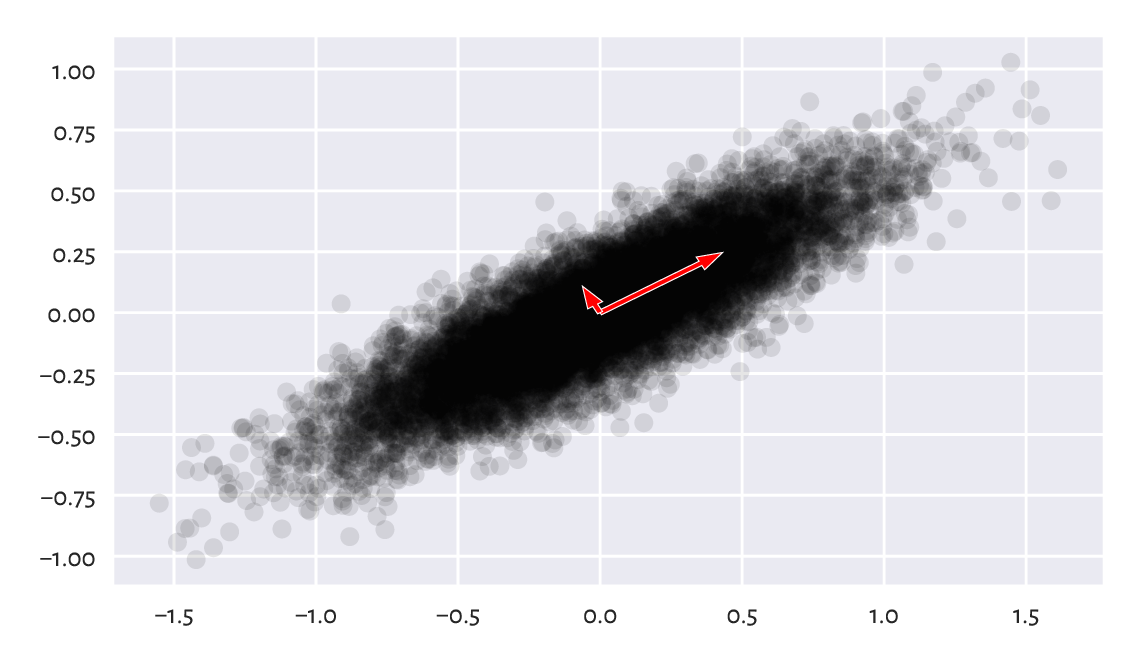Figure 9.18 Principal directions of an example dataset (scaled so that they are proportional to the standard deviations along them)

### 9.3.5. Dimensionality Reduction with SVD

Let us consider the following example three dimensional dataset.

chainlink = np.loadtxt("https://raw.githubusercontent.com/gagolews/" +


As we have told in Section 7.4, plotting is always done on a 2-dimensional surface (be it the computer screen or book page), therefore we can look at the dataset only from one angle at a time.

In particular, a scatterplot matrix only depicts the dataset from the perspective of the axes of the Cartesian coordinate system (standard basis), see Figure 9.19.

sns.pairplot(data=pd.DataFrame(chainlink))
# plt.show()  # not needed :/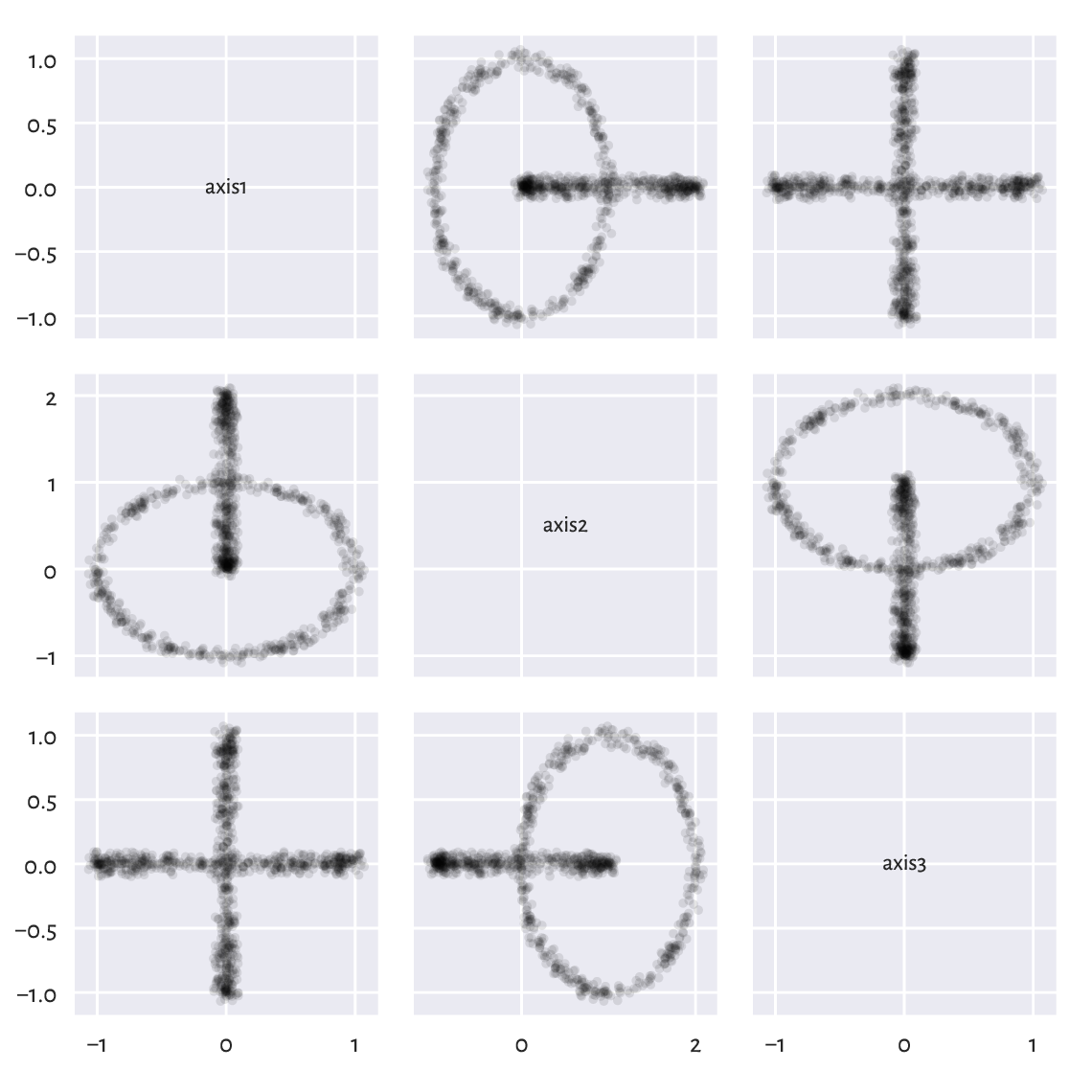Figure 9.19 Views from the perspective of the main axes

These viewpoints by no means must reveal the true geometric structure of the dataset. We know that we can actually rotate the virtual camera and find some more interesting angle. It turns out that our dataset represents two nonintersecting rings, hopefully visible Figure 9.20.

fig = plt.figure()
ax = fig.add_subplot(1, 3, 1, projection="3d", facecolor="#ffffff00")
ax.view_init(elev=45, azim=45, vertical_axis="z")
ax = fig.add_subplot(1, 3, 2, projection="3d", facecolor="#ffffff00")
ax.view_init(elev=37, azim=0, vertical_axis="z")
ax = fig.add_subplot(1, 3, 3, projection="3d", facecolor="#ffffff00")
ax.view_init(elev=10, azim=150, vertical_axis="z")
plt.show()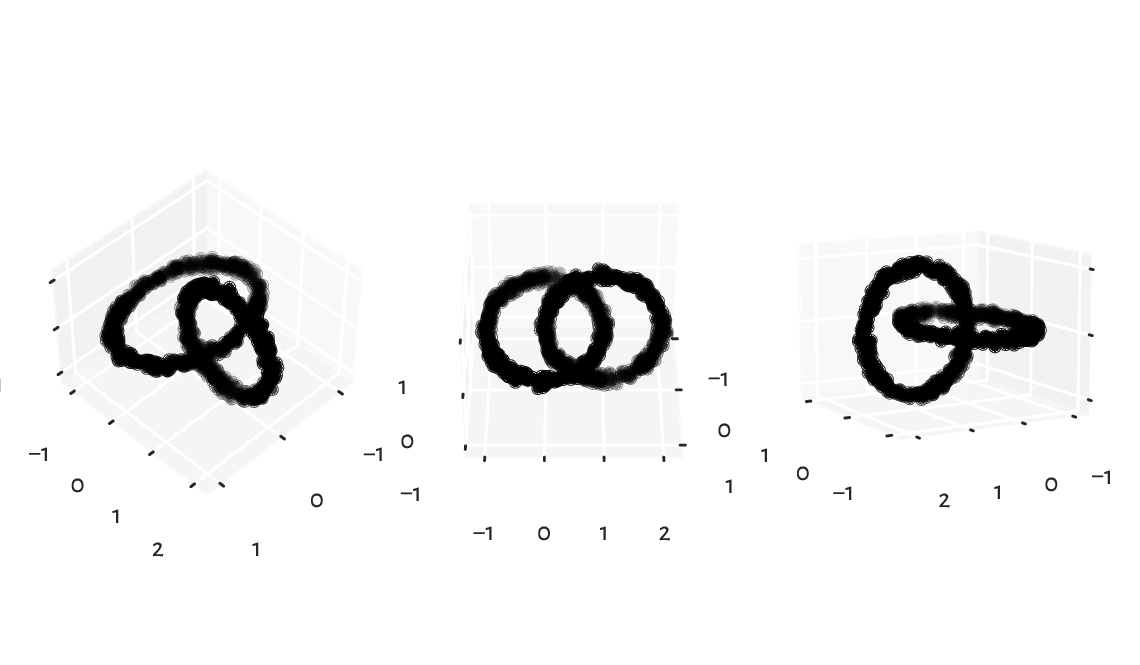Figure 9.20 Different views on the same dataset

It turns out that we may find (one of the many) such an noteworthy viewpoint using the SVD. Namely, we can perform the decomposition of a centred dataset which we denote with $$\mathbf{Y}$$:

$\mathbf{Y} = \mathbf{U}\mathbf{S}\mathbf{Q}$
import scipy.linalg
U, s, Q = scipy.linalg.svd(Y_centered, full_matrices=False)


Then, considering its rotated/reflected version

$\mathbf{T}=\mathbf{Y} \mathbf{Q}^{-1} = \mathbf{U}\mathbf{S}$

we know that its first column has the highest variance. Furthermore, the second column has the second highest variability, and so on. It might indeed be worth to look at that dataset from that most informative perspective.

Figure 9.21 gives the scatter plot for $$\mathbf{t}_{\cdot,1}$$ and $$\mathbf{t}_{\cdot,2}$$. Maybe this does not reveal the true geometric structure of the dataset (no single two-dimensional projection would do that here), but at least it is better than the initial ones (from the pairplot).

T2 = U[:, :2] @ np.diag(s[:2])  # the same as (U@np.diag(s))[:, :2]
plt.plot(T2[:, 0], T2[:, 1], "o")
plt.axis("equal")
plt.show()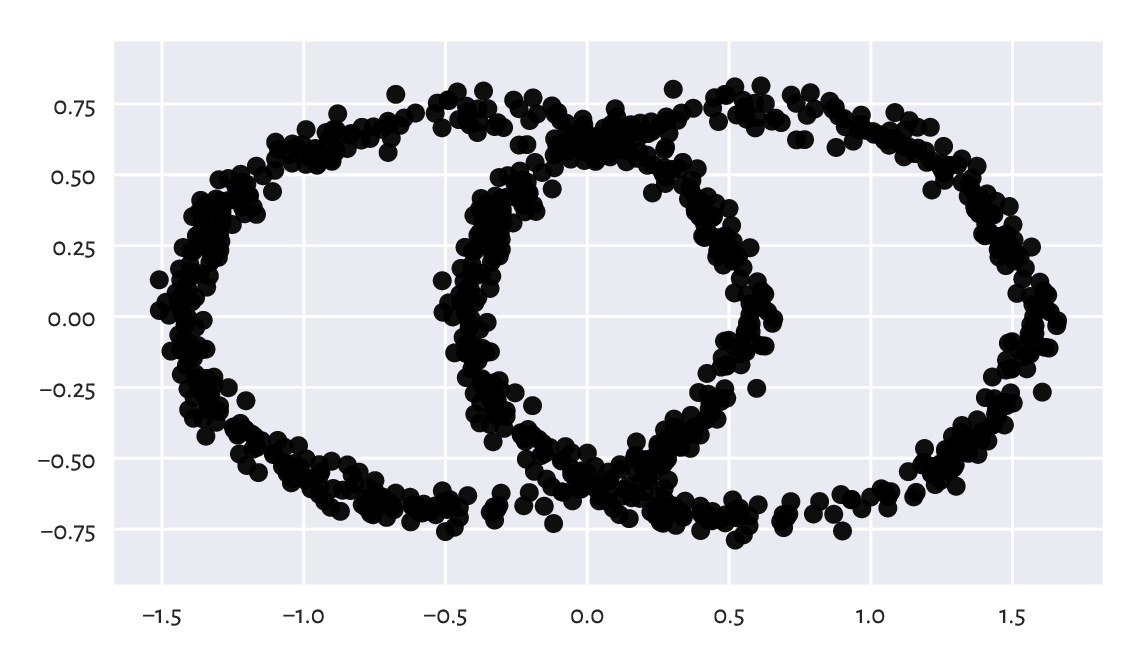Figure 9.21 The view from the two principal axes

What we have thus done was a kind of dimensionality reduction – we have found a viewpoint (in the form of an orthonormal matrix, being a mixture of rotations and reflections) on $$\mathbf{Y}$$ such that its orthonormal projection onto the first two axes of the Cartesian coordinate system is the most informative (in terms of having the highest variance, axiswisely).

Note

(**) The Eckart–Young–Mirsky theorem states that $$\mathbf{U}_{\cdot, :k} \mathbf{S}_{:k, :k} \mathbf{Q}_{:k, \cdot}$$ (where “:k” denotes “first k rows or columns”) is actually the best rank-$$k$$ approximation of $$\mathbf{Y}$$ with respect to both the Frobenius and spectral norms.

### 9.3.6. Principal Component Analysis

Principal component analysis (PCA) is a fancy name for the whole process of reflecting upon what happens along the projections onto the most variable dimensions. It can be used not only for data visualisation and deduplication, but also feature engineering (as in fact it creates new columns that are linear combinations of existing ones).

Let us consider a few chosen countrywise 2016 Sustainable Society Indices.

ssi = pd.read_csv("https://raw.githubusercontent.com/gagolews/" +
"teaching_data/master/marek/ssi_2016_indicators.csv",
comment="#")
Y = ssi.iloc[:, [3, 5, 13, 15, 19] ].to_numpy()
n = Y.shape
Y[:6, :]  # preview
## array([[ 9.32      ,  8.13333333,  8.386     ,  8.5757    ,  5.46249573],
##        [ 8.74      ,  7.71666667,  7.346     ,  6.8426    ,  6.2929302 ],
##        [ 5.11      ,  4.31666667,  8.788     ,  9.2035    ,  3.91062849],
##        [ 9.61      ,  7.93333333,  5.97      ,  5.5232    ,  7.75361284],
##        [ 8.95      ,  7.81666667,  8.032     ,  8.2639    ,  4.42350654],
##        [10.        ,  8.65      ,  1.        ,  1.        ,  9.66401848]])


Each index is on the scale from 0 to 10. These are, in this order:

1. Safe Sanitation,

2. Healthy Life,

3. Energy Use,

4. Greenhouse Gases,

5. Gross Domestic Product.

Above we have displayed the data corresponding to the 6 following countries:

countries = list(ssi.iloc[:, 0])
countries[:6]  # preview
## ['Albania', 'Algeria', 'Angola', 'Argentina', 'Armenia', 'Australia']


This is a 5-dimensional dataset, thus we cannot easily visualise it. That the pairplot does not reveal too much is left as an exercise.

Let us thus perform the SVD decomposition of a standardised version of this dataset (recall that centring is necessary, at the very least).

Y_std = (Y - np.mean(Y, axis=0))/np.std(Y, axis=0)
U, s, Q = scipy.linalg.svd(Y_std, full_matrices=False)


The standard deviations of the data projected onto the consecutive principal components (columns in $$\mathbf{U}\mathbf{S}$$) are:

s/np.sqrt(n)
## array([2.02953531, 0.7529221 , 0.3943008 , 0.31897889, 0.23848286])


It is customary to check the ratios of the variances explained by the consecutive principal components, which is a normalised measure of their importances. We can compute them by calling:

np.cumsum(s**2)/np.sum(s**2)
## array([0.82380272, 0.93718105, 0.96827568, 0.98862519, 1.        ])


Thus, we may say that the variability within the first two components covers 94% of the variability of the whole dataset. It might thus be a good idea to consider only a 2-dimensional projection of this dataset (actually, we are quite lucky here – or someone has selected these countrywise indices for us in a very clever fashion).

The rows in $$\mathbf{Q}$$ give us the so-called loadings. They give the coefficients defining the linear combinations of the rows in $$\mathbf{Y}$$ that correspond to the principal components.

Let us try to interpret them.

np.round(Q[0, :], 2)
## array([-0.43, -0.43,  0.44,  0.45, -0.47])


The first row in $$\mathbf{Q}$$ consists of similar values, but with different signs. We can consider them a scaled version of the average of Energy Use (column 3), Greenhouse Gases (4), and MINUS Safe Sanitation (1), MINUS Healthy Life (2), MINUS Gross Domestic Product (5). Possibly some kind of a measure of a country’s overall eco-unfriendliness?

np.round(Q[1, :], 2)
## array([ 0.52,  0.5 ,  0.52,  0.45, -0.02])


The second row in $$\mathbf{Q}$$ defines a scaled version of the average of Safe Sanitation (1), Healthy Life (2), Energy Use (3), and Greenhouse Gases (4), almost totally ignoring the GDP (5). Should we call it a measure of industrialisation? Something like this. But this naming is just for fun; mathematics – unlike the human brain – does not need our imperfect interpretations/fairy tales to function properly.

In Figure 9.22 is a scatter plot of the countries projected onto the said 2 principal directions. For readability, we only display a few chosen labels.

T2 = U[:, :2] @ np.diag(s[:2])  # == Y @ Q[:2, :].T
plt.plot(T2[:, 0], T2[:, 1], "o", alpha=0.1)
which = [   # hand-crafted/artisan
141, 117, 69, 123, 35, 80, 93, 45, 15, 2, 60, 56, 14,
104, 122, 8, 134, 128, 0, 94, 114, 50, 34, 41, 33, 77,
64, 67, 152, 135, 148, 99, 149, 126, 111, 57, 20, 63
]
for i in which:
plt.text(T2[i, 0], T2[i, 1], countries[i], ha="center") #countries[i])
plt.axis("equal")
plt.xlabel("1st principal component (eco-unfriendliness?)")
plt.ylabel("2nd principal component (industrialisation?)")
plt.show()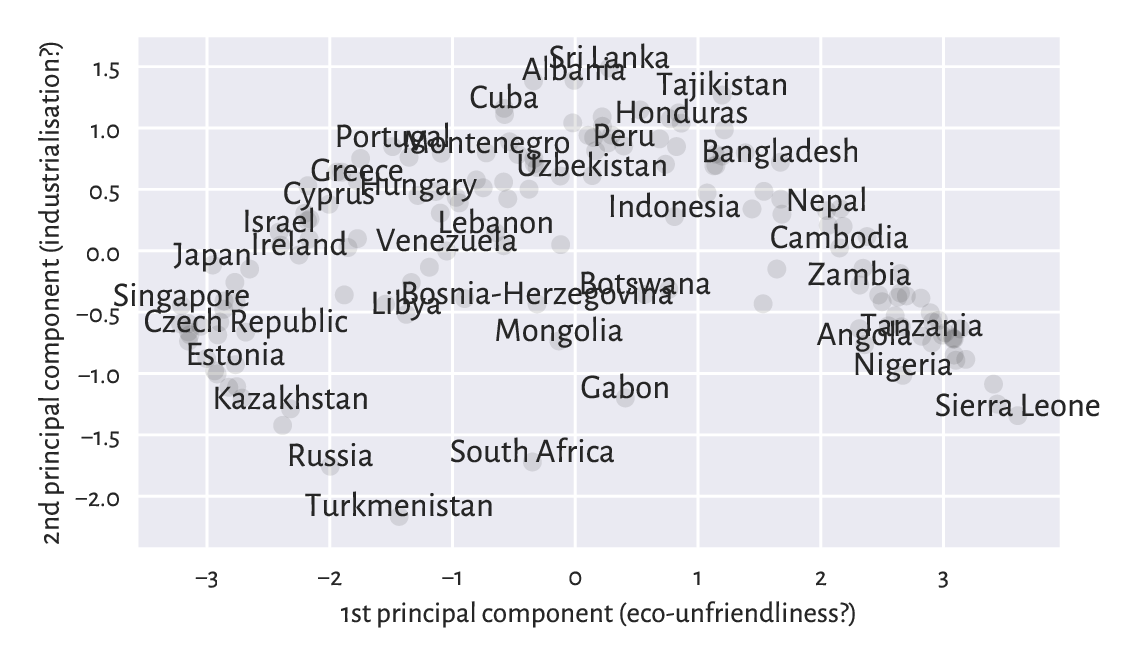Figure 9.22 An example principal component analysis of countries

This is merely a projection, but it might be an interesting one for some practitioners.

Some now-classic introductory text in statistical learning is [HTF17]. We recommend [Bis06, BHK20, DGL96] for more advanced students.

## 9.5. Exercises

Exercise 9.12

What does “correlation is not causation” mean?

Exercise 9.13

What does linear correlation of 0.9 mean? How about rank correlation of 0.9? And linear correlation of 0.0?

Exercise 9.14

How is the Spearman’s coefficient related to the Pearson’s one?

Exercise 9.15

How to write a linear model equation using the notion of the dot product?

Exercise 9.16

State the optimisation problem behind the least squares fitting of linear models.

Exercise 9.17

What are the different ways of the numerical summarising of residuals?

Exercise 9.18

Why is it important for the residuals to be homoscedastic?

Exercise 9.19

Is a more complex model always better?

Exercise 9.20

Why should extrapolation be handled with care?

Exercise 9.21

What is the difference between a train-test and a train-validate-test split?

Exercise 9.22

Why did we say that scikit-learn should be used carefully by novice users?

Exercise 9.23

What is a condition number of the model matrix and why it should always be checked?

Exercise 9.24

What is the geometrical interpretation of the dot product of two normalised vectors?

Exercise 9.25

How to verify if two vectors are orthogonal?

Exercise 9.26

What is an orthonormal projection? What is the inverse of an orthonormal matrix?

Exercise 9.27

What is the inverse of a diagonal matrix?

Exercise 9.28

Characterise the general properties of the three matrices obtained by performing the singular value decomposition of a given matrix of shape n-by-m.

Exercise 9.29

How to obtain the first principal component of a given centred matrix?

Exercise 9.30

How to compute the ratios of the variances explained by the consecutive principal components?

1

If a method Y is nothing else than X on transformed data, we should not consider it a totally new method.

2

And thus in order to memorise the model for a further reference, we only need to serialise its m coefficients, e.g., in a JSON or CSV file.

3

Due to computability and mathematical analysability, which we usually explore in more advanced courses on statistical data analysis, which this one is not.

4

In such an approach to regression, we are not aiming to minimise anything in particular. If the model is good with respect to some metrics such as RMSE or MAE, we can consider ourselves lucky. However, there are some asymptotic results that guarantee the optimality of the results generated (e.g., consistency) for large sample sizes, see, e.g., [DGL96].

5

For a model that is not generated via least squares, the coefficient of determination can also be negative, particularly when the fit is extremely bad. Also note that this measure is dataset-dependent. Therefore, it should not be used for comparing between models explaining different dependent variables.

6

Being themselves the square roots of eigenvalues of $$\mathbf{X}^T \mathbf{X}$$. Seriously, we really need linear algebra when we even remotely think about practising data science.